• #第三个参数为数据源 NBfit<-naiveBayes(as.factor(email$spam)~.,laplace=0,data=email) #用naiveBayes的结果做预测，第一个参数为用预测函数形成的对象，第二个参数为被预测的自变量的值 #再把预测好的应变量... 各种预测模型汇总 二、各种预测模型 先总结弄懂了的： 1、naiveBayes（第5课） #应变量y为email$spam，“~.”表示身下的所有属性都是自变量
#第二个参数我也不知道
#第三个参数为数据源
NBfit<-naiveBayes(as.factor(email$spam)~.,laplace=0,data=email) #用naiveBayes的结果做预测，第一个参数为用预测函数形成的对象，第二个参数为被预测的自变量的值 #再把预测好的应变量的值存入对象pred2中 pred2<-predict(NBfit,email[,2:19]) #比较预测值与真实值的差别 #形成一个2*2的矩阵，对角线为正确的，其余为预测错误的 table(pred2, email$spam)

2、线性回归（lm函数）-第4课
#createDataPartition函数用于制作训练集，其中y=faithful$waiting指按照该属性分类，p=0.5指将p*100%的值用于训练，list=FALSE不用管，默认为false inTrain<-createDataPartition(y=faithful$waiting, p=0.5, list=FALSE)
#其中50%用于训练
trainFaith<-faithful[inTrain,]
#剩下50%用于测试
testFaith<-faithful[-inTrain,]

#回归模型函数在这里################################
#同上，y~x，数据源
lm1<-lm(eruptions~waiting, data=trainFaith)

newdata<-data.frame(waiting=80)
predict(lm1, newdata)

注：由于lm函数的预测值是一个置信度为大致0.95左右（可以自己定义）的区间，所以predict共有3个属性结果，所以不可以用它来预测table之类的混淆矩阵
3、决策树：（rpart函数）
# grow the tree
fit <- rpart(Kyphosis ~ Age + Number + Start,
method="class", data=kyphosis)

printcp(fit) # display the results
plotcp(fit) # visualize cross-validation results
summary(fit) # detailed summary of splits

# plot tree
rpart.plot(fit,extra=106, under=TRUE, faclen=0,
cex=0.8, main="Decision Tree")

# prediction
result <- predict(fit,kyphosis[,-1],type="class")

# confusing matrix
table(pred = result, true = kyphosis[,1])

以下左图为可视化交叉验证结果，右图为系统做出的决策树4、剪枝数（是决策树的一种，也是rpart函数，只不过参数中多了一个control = rpart.control(minsplit = 10)约束，其中minsplit表示：试图进行分割时，节点中必须存在的最小观测数。）
# prune the tree
# minsplit: the minimum number of observations that must exist in a node in order for a split to be attempted.
pfit1 <- rpart(Kyphosis ~ Age + Number + Start,
method="class", data=kyphosis,
control = rpart.control(minsplit = 10))

# plot the pruned tree
rpart.plot(pfit1,extra=106, under=TRUE, faclen=0,
cex=0.8, main="Decision Tree")
#prediction
result<-predict(pfit1,kyphosis[,-1],type="class")
# confusing matrix
table(pred = result, true = kyphosis[,1])

5、回归树（也是rpart函数的一种，只不过method=“anova”，只有这点不同）-第7课
fit <- rpart(Mileage~Price + Country + Reliability + Type,
method="anova", data=cu.summary)


6、随机森林（先用n棵树预测，再将这n棵树的结果投票选出最优预测，权重相同，同时进行）-第8课
## Random Forest model
# mtry is the number of variables to try这里的ntree=100就是说要用100棵树先同时预测
fit <- randomForest(y ~ .,   data=train, mtry=4, ntree=100)
print(fit) # view results
importance(fit) # importance of each predictor
varImpPlot(fit)

# prediction resutls
RandomTreeresult<-predict(fit,test[,-17],type="class")
# confusing matrix
table(pred = RandomTreeresult, true = test$y)  7、adaboost森林（依次进行不同的预测树，每一次错误时，将权重增大，正确时将权重减小，下一次的值由上一次的值递推得到）-第8课 eg： adaboost<-boosting(y~., data=train, boos=FALSE, mfinal=20,coeflearn='Breiman') summary(adaboost) adaboost$trees
adaboost$weights adaboost$importance

# error
plot(errorChange$error,type='l') # peformance on adTest Data adpred<-predict(adaboost,test) table(pred=adpred$class,true=test$y) # Trees Visulizations: T1,T2, T19, T20 t1<-adaboost$trees[]
t2<-adaboost$trees[] rpart.plot(t1,under=TRUE, faclen=0, cex=1, main="Decision Tree 1") rpart.plot(t2,under=TRUE, faclen=0, cex=1, main="Decision Tree 2")  2、逻辑回归（Logistic regression）（第5课） 是一种线性的回归 #线性回归分析常用glm函数——逻辑回归 #第一个参数的格式为：y~x，y为应变量，x为自变量，当x为.时，自变量取除了y以外的所有属性 #第二个参数为数据源，第三个参数表示预测集为二元 g_full=glm(spam~ ., data=email, family="binomial") #接下来进行预测 #confusionMatrix函数的第一个参数表示预测的向量，第二个表示真实的向量 #这个函数的作用是①列出table ②分析精准度等等参数 #由于predict函数预测出来的结果是实数，而这里只需要0/1即可，所以要用ifelse来制约一下 pred<-ifelse(predict(g_full, email[,2:19])>0.5,1,0) acc <- confusionMatrix(as.factor(pred), as.factor(email$spam))
acc

3、KNN（K Nearest Neighbor Classifiers）（第5课） 维度很高时不适用，运算过程太耗费资源 （想象一个圆圈用于分类不同类型）
# normalize
#标准化
iris_new<-scale(iris_random[,-5],center = TRUE,scale = TRUE)

# data visulization
# 划散点图
ggplot(aes(iris_random$Sepal.Length, iris_random$Petal.Width), data = iris_random)+
geom_point(aes(color= factor(iris_random$Species))) # constrcut training and testing mannually #抽出训练和测试数据集 train <- iris_new[1:100,] test <- iris_new[101:150,] train_sp <- iris_random[1:100,5] test_sp <- iris_random[101:150,5] # knn训练 model <- knn(train= train,test=test,cl= train_sp,k=8) model #汇总统计分布 table(factor(model)) #真实和预测的分布 table(test_sp,model) #choose the right k#### #First Attempt to Determine Right K #### #存储准确率 iris_acc<-numeric() #Holding variable #k从1-50取值，看哪一个准确率高 for(i in 1:50){ #Apply knn with k = i predict<-knn(train= train,test=test,cl= train_sp,k=i) iris_acc<-c(iris_acc, mean(predict==iris_random[101:150,5])) } #Plot k= 1 through 50 #画图，准确率和k值的关系 plot(1-iris_acc,type="l",ylab="Error Rate", xlab="K",main="Error Rate for Iris With Varying K") # 从图像中看出k= 12 时最优 # optimal k =12 model <- knn(train= train,test=test,cl= train_sp,k=12) table(test_sp,model)  4、perceptron（是一种线性分类器） ##iris DATA #鸢尾花的那些数据，实验方法和上面的例子相同 data(iris) pairs(iris[1:4], main = "Anderson's Iris Data -- 3 species", pch = 21, bg = c("red", "green3", "blue")[unclass(iris$Species)])

# select the Sepal.Width versus Petal.Width scatter plot
x <- cbind(iris$Sepal.Width,iris$Petal.Width)
# label setosa as positive and the rest as negative
Y <- ifelse(iris$Species == "setosa", +1, -1) # # plot all the points plot(x,cex=0.5) # use plus sign for setosa points points(subset(x,Y==1),col="black",pch="+",cex=2) # use minus sign for the rest points(subset(x,Y==-1),col="red",pch="-",cex=2) p <- perceptron(x,Y) plot(x,cex=0.2) points(subset(x,Y==1),col="black",pch="+",cex=2) points(subset(x,Y==-1),col="red",pch="-",cex=2) # compute intercept on y axis of separator # from w and b intercept <- - p$b / p$w[] # compute slope of separator from w slope <- - p$w[] /p$w[] # draw separating boundary abline(intercept,slope,col="red")  5、SVM（Support vector machines） #subset 1: setosa and virginica #把其中两种花区分出来（易区分） iris.part1 = subset(iris, Species != 'versicolor') pairs(iris.part1[,1:4], col=iris.part1$Species)
iris.part1$Species = factor(iris.part1$Species)

#subset 2: versicolor and virginica
#把另外两种花区分出来（不易区分）
iris.part2 = subset(iris, Species != 'setosa')
pairs(iris.part2[,1:4], col=iris.part2$Species) iris.part2$Species = factor(iris.part2$Species) iris.part1 = iris.part1[, c(1,2,5)] iris.part2 = iris.part2[, c(1,2,5)] # linear #线性可分的情况 plot(iris.part1$Sepal.Length,iris.part1$Sepal.Width,col=iris.part1$Species)

fit1 = svm(Species ~ ., data=iris.part1, type='C-classification')
plot(fit1, iris.part1)
fit1.pred<-predict(fit1,iris.part1[,-3])
table(pred = fit1.pred, true = iris.part1[,3])

# linear
#部分线性不可分的情况
fit2 = svm(Species ~ ., data=iris.part2, type='C-classification', kernel='linear')
plot(fit2, iris.part2)
fit2.pred<-predict(fit2,iris.part2[,-3])
table(pred = fit2.pred, true = iris.part2[,3])

# Non-linear
#线性不可分
plot(iris.part2$Sepal.Length,iris.part2$Sepal.Width,col=iris.part2$Species) fit3 = svm(Species ~ ., data=iris.part2, type='C-classification', kernel='radial') plot(fit3, iris.part2) fit3.pred<-predict(fit3,iris.part2[,-3]) table(pred = fit3.pred, true = iris.part2[,3]) # multiple-class #多类别的判定 svm_model <- svm(Species ~ ., data=iris) summary(svm_model) x <- subset(iris, select=-Species) y <- iris$Species

fit4.pred<-predict(svm_model,x)
table(pred = fit4.pred, true = y)

plot(svm_model, iris, Petal.Width ~ Petal.Length,
slice = list(Sepal.Width = 3, Sepal.Length = 4))

7、拉索回归和ridge回归-第4课（没看懂，mark）
预测方式、方法
1、将二元的y值预测强制转换
##因为predict函数预测出来的值是实数，而结果值只有1、0，这样一来就
##需要ifelse函数用于制约y值了，当大于0.5时y值为1，否则为0
pred<-ifelse(predict(g_full, email[,2:19])>0.5,1,0)

2、混淆矩阵 ①table 该函数的两个参数分别为预测的所有y值，和真实的所有y值
table(pred2, email$spam)  ② confusionMatrix函数，参数和table一致，只是需要将格式更改为factor才可行 acc <- confusionMatrix(as.factor(pred2), as.factor(email$spam))
acc

展开全文决策树
• 预测分析数据挖掘项目 要查看我的项目，请单击下面的图像 :backhand_index_pointing_down:
• 所以这里通过墨尔本十年气温变化预测的任务来整理一个时间序列数据挖掘的模板，方便以后查阅方便。这个模板可以用在大部分的时间序列预测任务，从股票价格波动，到四季气温变化， 从大桥沉降预测，到城市用电预警等...
1. 写在前面
本篇文章主要是时间序列数据挖掘与机器学习：墨尔本十年气温数据集视频的学习笔记， 详细内容还请移步B站子豪兄的视频吧。
时间序列数据广泛存在于量化交易， 回归预测等机器学习应用， 是最常见的数据类型。所以这里通过墨尔本十年气温变化预测的任务来整理一个时间序列数据挖掘的模板，方便以后查阅方便。这个模板可以用在大部分的时间序列预测任务，从股票价格波动，到四季气温变化， 从大桥沉降预测，到城市用电预警等。
要进行下面工作的整理：
探索性数据挖掘和数据可视化： 绘制折线图、热力图、箱型图、小提琴图、滞后图、自相关图， 让枯燥的时间序列数据颜值爆表！对时间做特征工程：扩展时间数据维度，这个代码模板也可以作为时间序列数据的通用预处理模板使用多种机器学习模型建立回归拟合模型： 线性回归、多项式回归、岭回归、随机森林、神经网络等，并可视化展示多种模型效果进行对比
通过这次整理，掌握sklearn中常用的工具包以及深度神经网络的搭建Keras，能够学习到处理时间序列的方式，里边还包含了大量的数据可视化的套路。
由于篇幅原因，这里可能不会把所有的代码执行结果展示出来， 只会展示比较重要的结果，毕竟为了以后方便查阅，太多结果图像在反而不太好。
OK， let’s go!
2. 导入包和墨尔本1980-1990十年气温数据集
# Python的数据处理库pandas，类似Excel
import pandas  as pd

# Python绘图工具
import matplotlib.pyplot as plt
import seaborn as sns   # 这个是matplotlib的进一步封装，绘制的图表更加高大上
%matplotlib inline

# 设置绘图大小
plt.style.use({'figure.figsize':(25, 20)})

plt.rcParams['font.sans-serif'] = ['SimHei']  # 用来正常显示中文标签
plt.rcParams['axes.unicode_minus'] = False  # 用来正常显示负号

import warnings  # 过滤警告用
warnings.filterwarnings('ignore')

下面导入墨尔本气温数据集
"""读取数据集"""

3. 数据探索
3.1 探索性分析初步
第一列Date为日期，也就是时间序列，我们将第一列的数据类型转换为pandas中的datatime日期类型，并将这一列作为数据的索引，便于后续处理。
df['Date'] = pd.to_datetime(df['Date'])
df = df.set_index('Date')

"""df是pandas里面的DataFrame数据类型，具有一系列封装好的属性和方法。  .后面带()是方法，不带()的是属性"""

然后数据简单探索
# 再查看一下数据
# 查看各列信息
df.info()
# 统计信息
df.describe()

3.2 数据的EDA分析
下面是数据的EDA分析pandas_profiling直接生成数据报告，这个是比赛探索数据神器，在这里面会看到：
总体的数据信息（首先是数据集信息：变量数(列)、观察数(行)、数据缺失率、内存；数据类型的分布情况），  警告信息

要点：类型，唯一值，缺失值分位数统计量，如最小值，Q1，中位数，Q3，最大值，范围，四分位数范围描述性统计数据，如均值，模式，标准差，总和，中位数绝对偏差，变异系数，峰度，偏度
单变量描述（对每一个变量进行描述）  相关性分析（皮尔逊系数和斯皮尔曼系数）  采样查看等
import pandas_profiling as ppf
ppf.ProfileReport(df)

3.3 可视化一些特征
这里会通过各种图进行展示数据的特征，重点是这些图怎么使用。
3.3.1 折线图
我们把Temp，也就是温度属性用折线图可视化一下：
"""绘制折线图"""
df['Temp'].plot(figsize=(30,15))
# 设置坐标字体大小
plt.tick_params(labelsize=30)
# 生成刻度线网格
plt.grid()

"""折线图容易产生毛刺，可以换成散点图"""

看一下结果：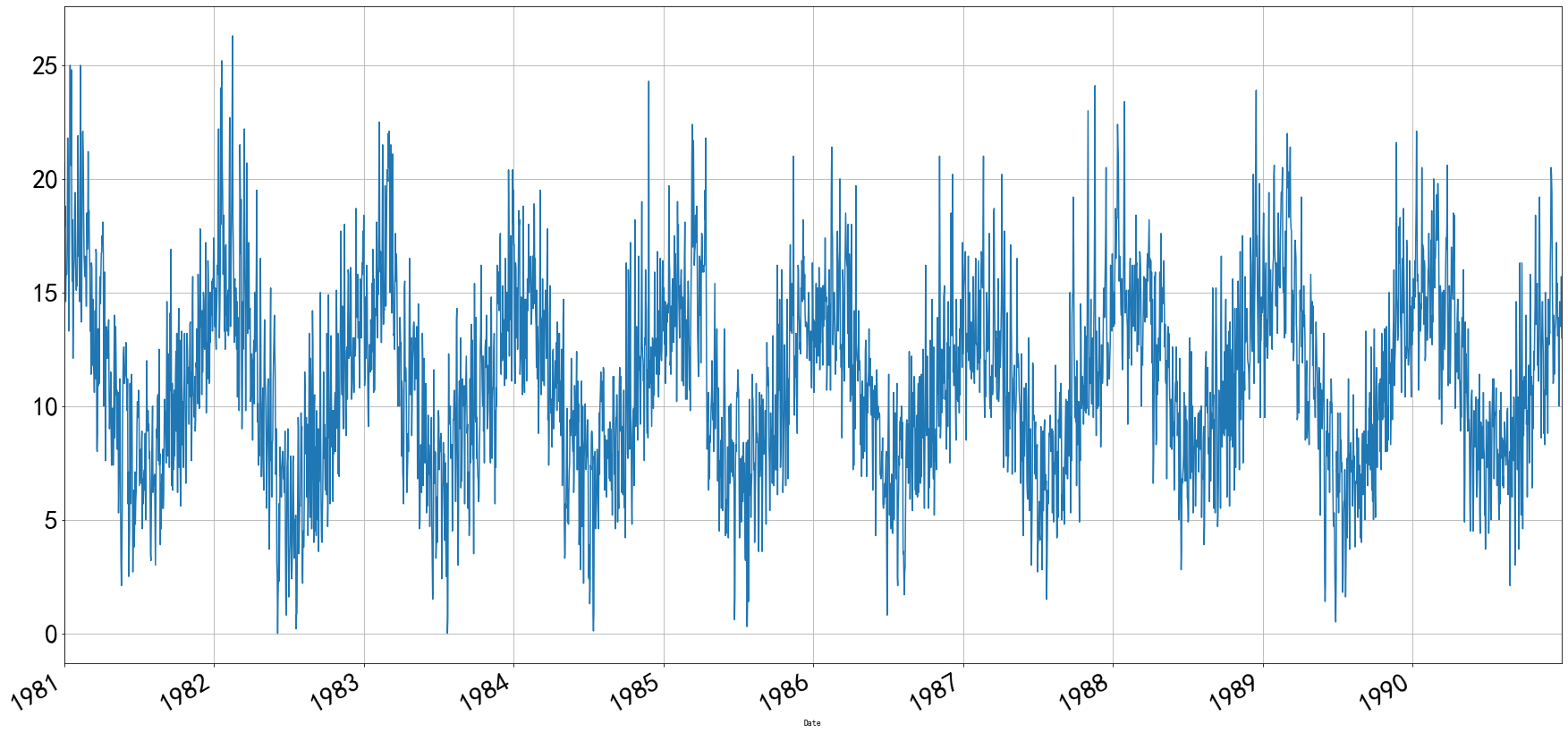3.3.2 散点图
"""绘制散点图"""
df['Temp'].plot(style='k.', figsize=(30,15))
# 设置坐标字体大小
plt.tick_params(labelsize=30)
# 生成刻度线网格
plt.grid()

结果：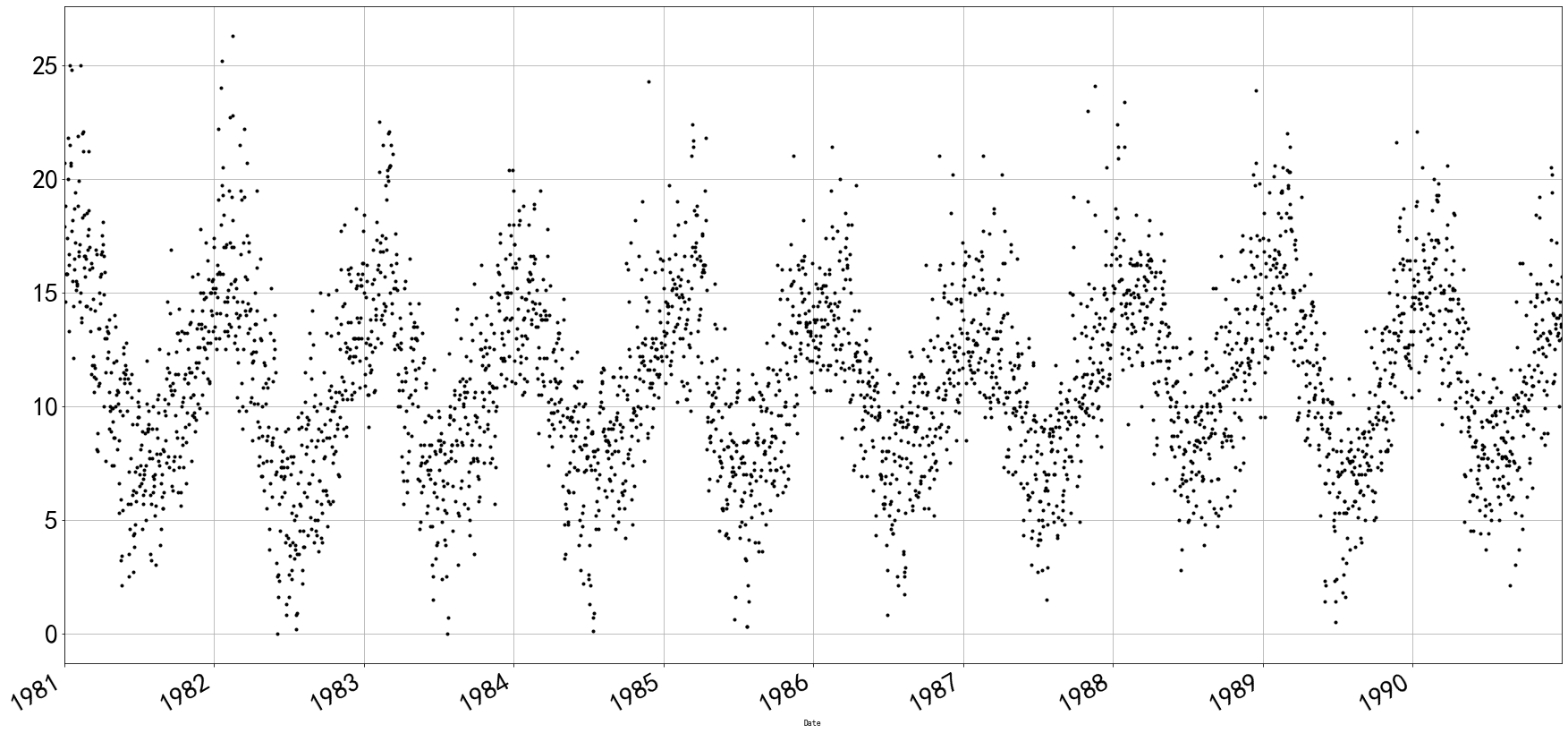3.3.3 直方图
# 设置绘图大小
plt.style.use({'figure.figsize':(5, 5)})
df['Temp'].plot(kind='hist', bins=20)  # bins参数表示横轴多少等分

# 还有一种
df['Temp'].hist(bins=50)

结果：3.3.4 堆积面积图
# 设置绘图大小
plt.style.use({'figure.figsize':(10, 5)})
df.plot.area(stacked=False)

结果：3.3.5 核密度估计图（KDE图）
# 设置绘图大小
plt.style.use({'figure.figsize':(10, 5)})
df['Temp'].plot(kind='kde')

结果：3.3.6 热力图
将1982年每一个月的最低气温用热力图展示出来 关于pandas的重采样resample
# 设置绘图大小
plt.style.use({'figure.figsize':(20, 8)})

df['1982'].resample('M').mean().T

sns.heatmap(df['1982'].resample('M').mean().T)

"""为什么6月和7月气温最低？  因为墨尔本在澳大利亚，属于南半球，6月和7月是冬天"""

结果：3.4 绘制每年气温变化的直线图，箱型图，热力图，小提琴图
我们刚刚将日期列转换成了pandas中的datetime类型，我们可以直接通过年份和日期索引选择指定时间的数据
df['1984']

下面实用pandas的groupby操作，把每年和每天的温度筛选出来python处理数据的风骚操作pandas 之 groupby&agg
groups = df.groupby(pd.Grouper(freq='1Y'))['Temp']
years = pd.DataFrame()
for name, group in groups:
years[name.year] = group.values

years

这一个要看一下实现了什么效果：这个就是把十年的每一天的数据都给做了出来，每一列代表年，每一行是天。下面绘制图像进行可视化上面这个表：
# 设置绘图大小
plt.style.use({'figure.figsize':(30, 15)})

years.plot()

# 设置图例文字大小和图示大小
plt.legend(fontsize=15, markerscale=15)
# 设置坐标文字大小
plt.tick_params(labelsize=15)

结果：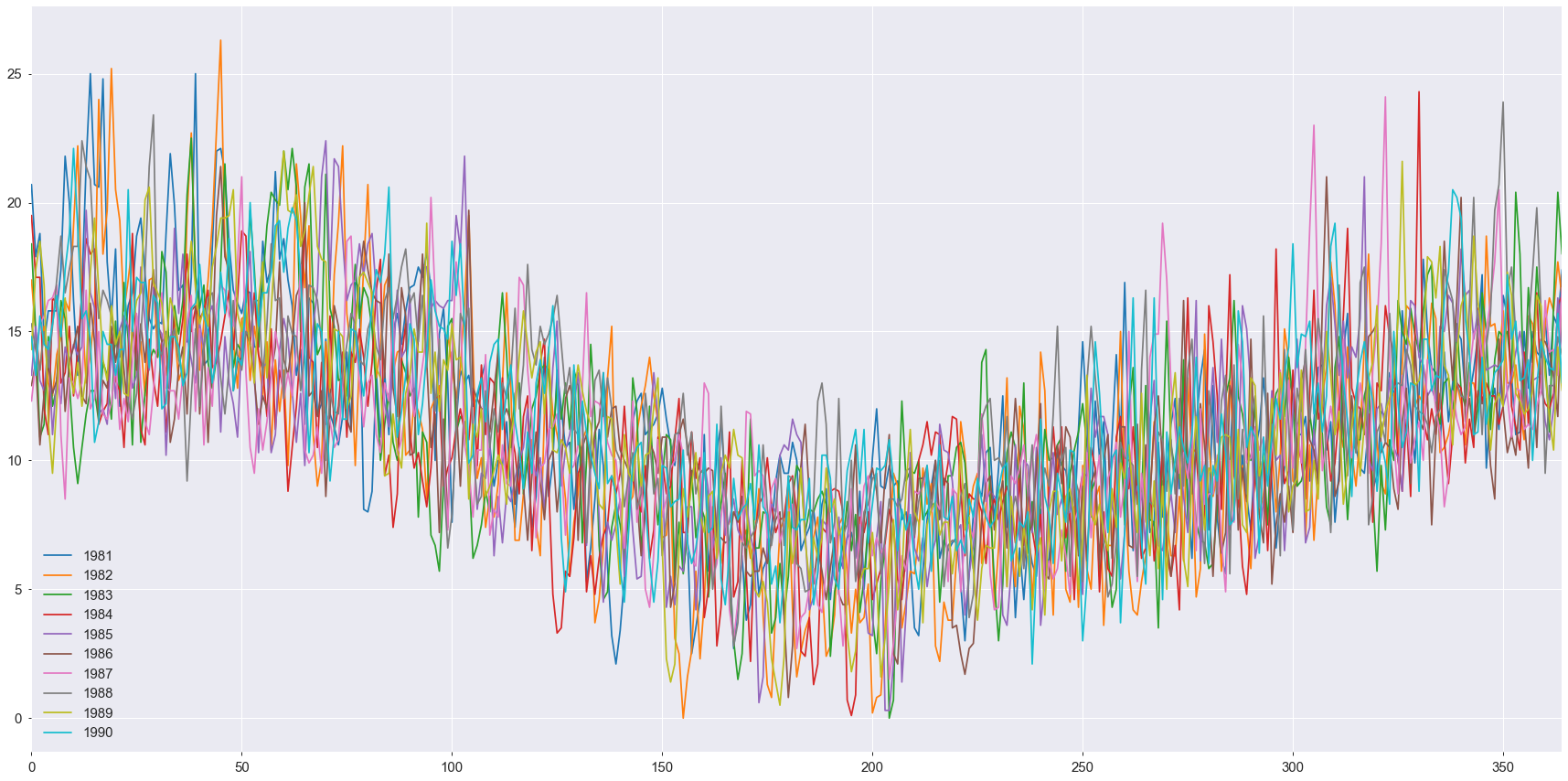3.4.1 折线图
"""折线图"""
years.plot(subplots=True, figsize=(20, 45))
plt.show()

这个会绘制每一年的折线图，结果就不全显示了：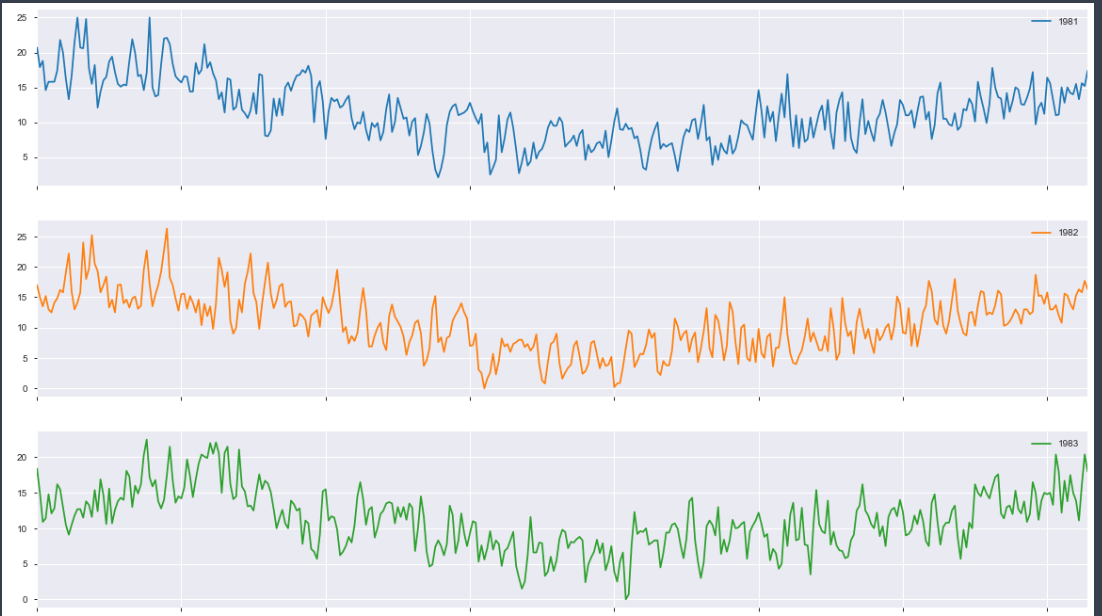3.4.2 箱型图
这个图可以很容易的看出离群点和数据的分布
"""箱型图"""
years.boxplot(figsize=(20, 10))

结果如下：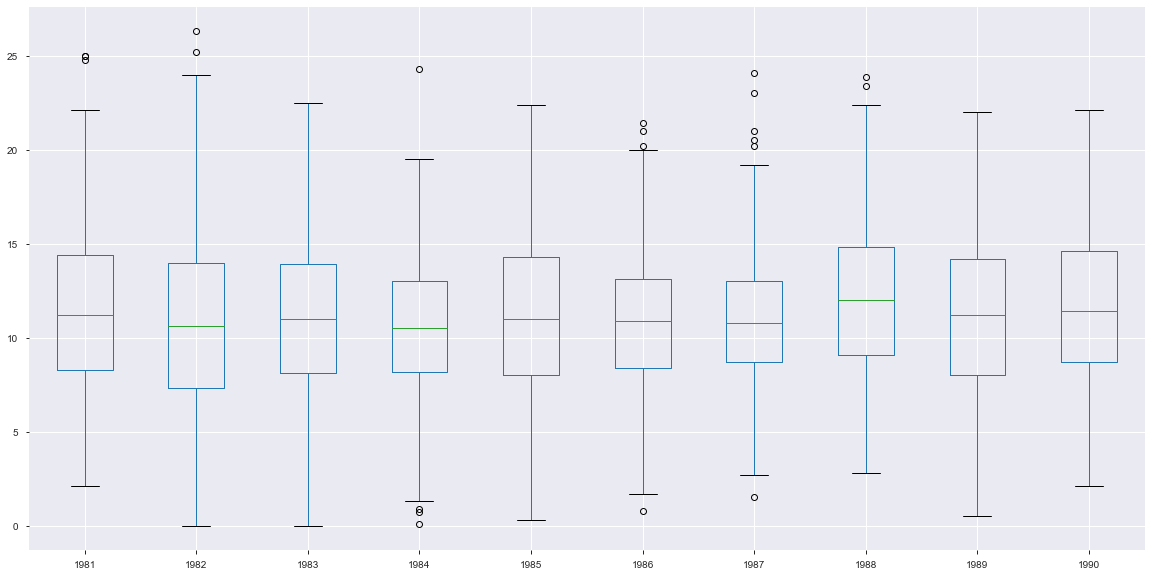3.4.3 热力图
# 设置绘图大小
plt.style.use({'figure.figsize':(30, 10)})
sns.heatmap(years.T)
"""颜色越黑，表示温度越低， 颜色越亮，表示温度越高"""

结果：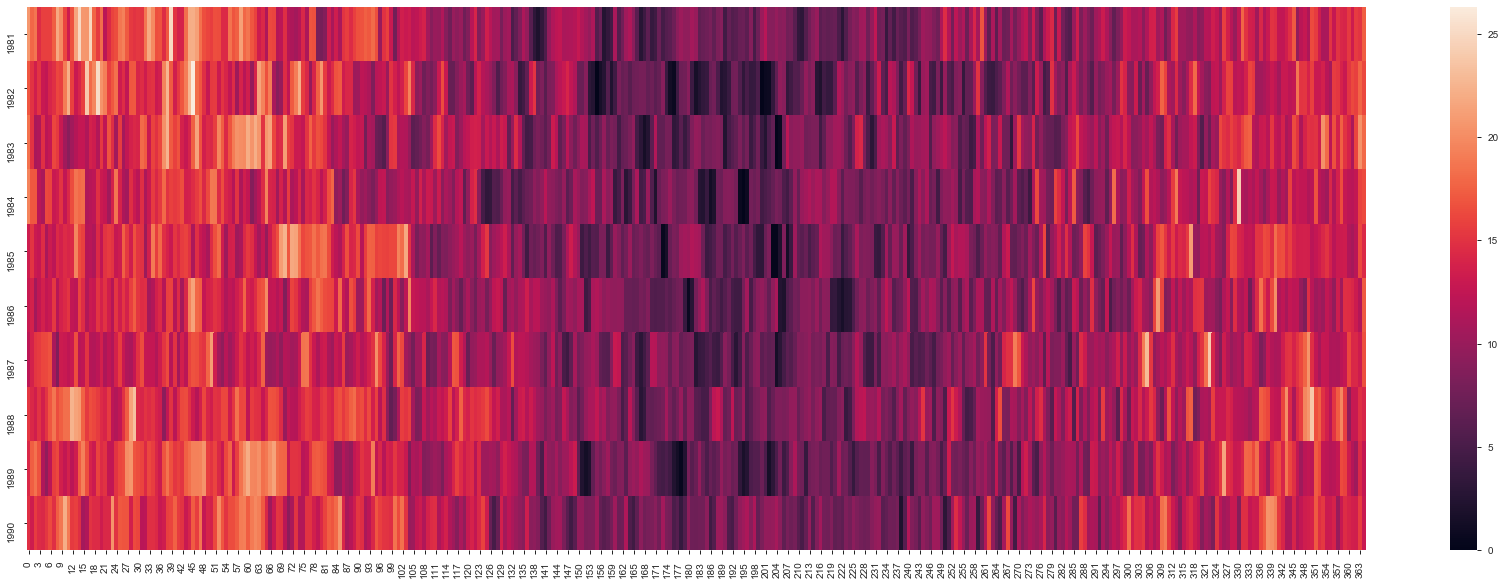plt.matshow(years.T, interpolation=None, aspect='auto')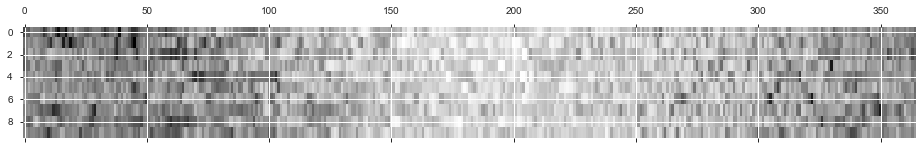3.4.4 每一年气温的直方图
"""每一年气温的直方图"""
plt.style.use({'figure.figsize':(30, 22)})
years.hist(bins=15)

这个不做展示。
下面绘制1985年12个月每天的气温数据，这个和上面的异曲同工，只不过那个是每一年的每一天，这个是每一个月的每一天。
# 选取1985年12个月每天的气温数据
groups_month = df['1985'].groupby(pd.Grouper(freq='1M'))['Temp']

months = pd.concat([pd.DataFrame(x.values) for x in groups_month], axis=1)
months = pd.DataFrame(months)
months.columns = range(1, 13)
months

看一下months：绘制箱型图：
months.boxplot(figsize=(20, 15))
plt.title('墨尔本1982年每个月最低温度分布箱型图')

绘制热力图：
# 设置图像大小
plt.style.use({'figure.figsize':(5, 8)})
sns.heatmap(months)
plt.title('墨尔本1982年每天最低气温分布热力图')

plt.matshow(months, interpolation=None, aspect='auto', cmap='rainbow')

3.4.5 小提琴图
类似箱型图，只不过比箱型图更加高级一些
# 设置图像大小
plt.style.use({'figure.figsize':(15, 10)})

sns.violinplot(data=months)
plt.title('墨尔本1982年每个月最低气温分布小提琴图')

# linewidth参数可以控制线宽
sns.violinplot(data=months, linewidth=3)
plt.title('墨尔本1982年每个月最低气温分布小提琴图')

结果：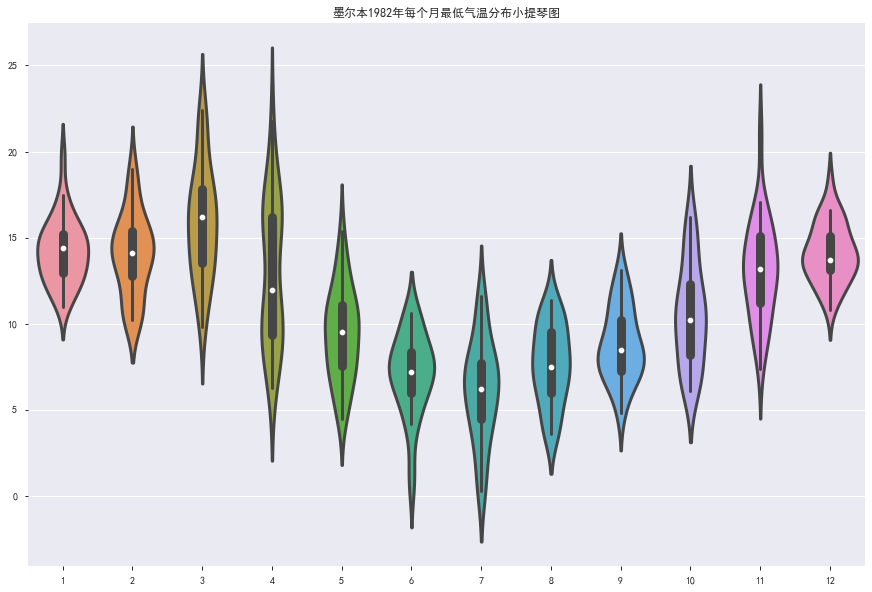3.5 滞后散点图
时间序列分析假定一个观测值与前面的观测值之间存在一定的关系。 相对于某观察值之前的观测值被称为滞后值，在一个时间步长前的观测值称为滞后一期，在两个时间步长前的观测值称为滞后二期，依次类推。 比如， 对于1982年8月15日的气温数据，8月14日的气温为滞后一期，8月13日的气温为滞后二期 每个观察值之间和其滞后值之间的关系，可以用滞后散点图表示
from pandas.plotting import lag_plot

# 设置图像大小
plt.style.use({'figure.figsize':(10, 10)})

lag_plot(df['Temp'])
plt.title('墨尔本1980-1990年最低气温滞后1期散点图')

结果：散点图聚在左下角到右上角，表示与滞后值正相关。 散点图聚在左上角到右下角，表示与滞后值负相关。 离对角线越紧密，表示相关关系越强。 分散的球状散点图表示相关关系微弱。
横轴表示每天的气温，纵轴表示滞后一天的气温
通过lag参数控制滞后值
lag_plot(df['Temp'], lag=3)
plt.title('墨尔本1980-1990年最低气温滞后3期散点图')

结果：还可以绘制不同滞后值对应的散点图：
"""绘制不同滞后值对应的滞后散点图"""
lag_list = [1, 2, 3, 5, 10, 20, 50, 100, 150, 180]
plt.style.use({'figure.figsize':(15, 35)})
for i in range(len(lag_list)):
ax = plt.subplot(5, 2, i+1)
ax.set_title('t vs t+' + str(lag_list[i]))
lag_plot(df['Temp'], lag=lag_list[i])
plt.title('墨尔本1980-1990年最低气温滞后{}期散点图'.format(lag_list[i]))

3.6 自相关图
这个图还是比较重要的，尤其是对于时间序列来说，可以看出周期和相关性。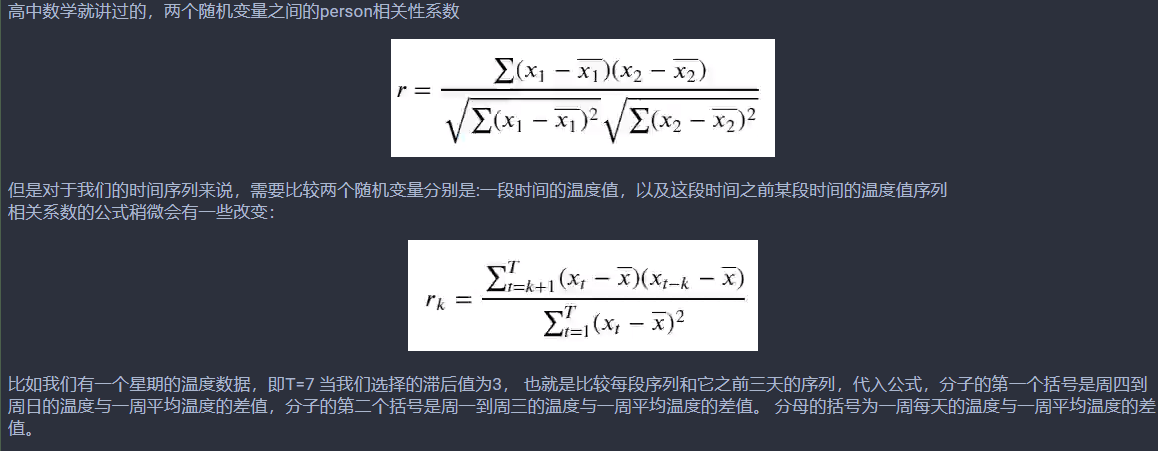代码如下：
from pandas.plotting import autocorrelation_plot

# 设置图像大小
plt.style.use({'figure.figsize':(15, 10)})

autocorrelation_plot(df['Temp'])
plt.title('墨尔本1980-1990最低气温自相关图')

# 设置坐标文字大小
plt.tick_params(labelsize=10)
plt.yticks(np.linspace(-1, 1, 120))

结果如下：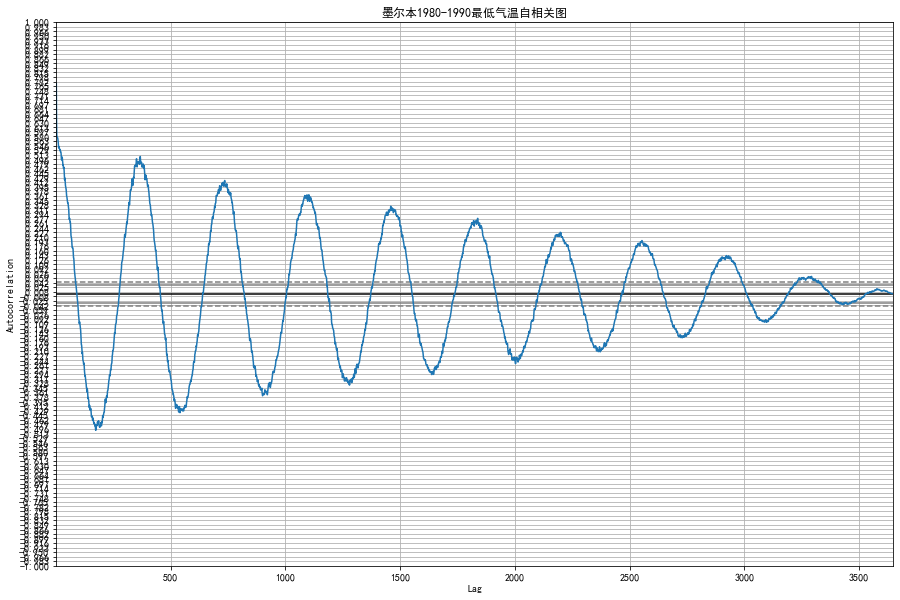比如说横轴是1000， 就表示这个数据集所有数据与它之后1000天的数据的自相关程度。
时间间隔的越远，温度之间的自相关程度就摆动着降低。 并且在时间向远推进的过程中，还会出现周期性的波动，这是由季节的周期性更迭造成的
如果我们随机取点绘制滞后图和自相关图，就会发现完全没有规律。
# 设置图像大小
plt.style.use({'figure.figsize':(10, 5)})

a = np.random.randn(100)
a = pd.Series(a)
lag_plot(a)
plt.title("随机数列的1期滞后散点图")

结果：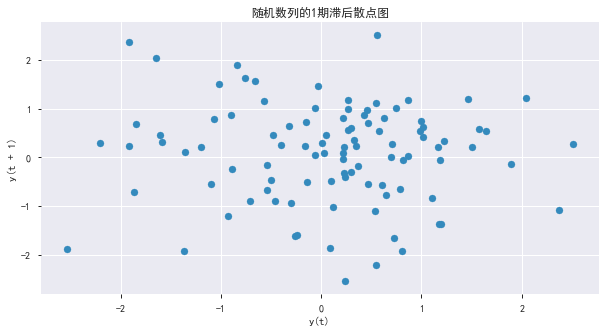autocorrelation_plot(a)
plt.title('随机数列的自相关图')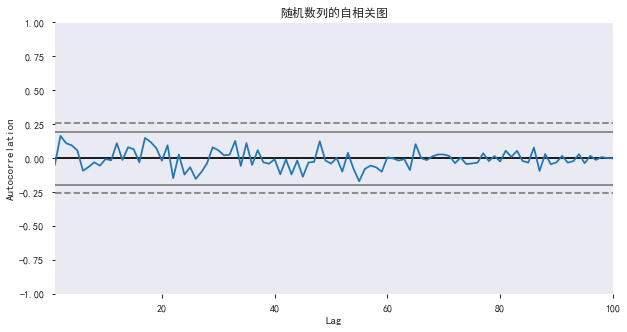4. 时间数据的特征工程
将单纯的日期维度扩展成更多维度，构造更多的输入给模型的特征，增强数据的可解释性，更接近人的业务逻辑。
哪一年哪一个月星期几这个月的第几天月初还是月末一年当中的第几天一年当中的第几个月
# 重新调用pandas的read_csv函数读取数据集文件
df2['Date'] = pd.to_datetime(df2['Date'])

下面开始构造数据特征：
把年月日作为特征构造成列 # 构造新的一列： 年
df2.loc[:, 'year'] = df2['Date'].apply(lambda x: x.year)

# 构造新的一列： 月
df2.loc[:, 'month'] = df2['Date'].apply(lambda x: x.month)

# 构造新的一列： 星期几
df2.loc[:, 'dow'] = df2['Date'].apply(lambda x:x.dayofweek)

# 构造新的一列： 一个月第几天
df2.loc[:, 'dom'] = df2['Date'].apply(lambda x: x.day)
  是不是周末，是不是周六，是不是周日 # 构造新的三列： 是不是周末、是不是周六、是不是周日
df2.loc[:, 'weekend'] = df2['Date'].apply(lambda x:x.dayofweek > 4)
df2.loc[:, 'weekend_sat'] = df2['Date'].apply(lambda x: x.dayofweek == 5)
df2.loc[:, 'weekend_sun'] = df2['Date'].apply(lambda x: x.dayofweek == 6)
  添加上半月和下半月的信息
# 添加上半月和下半月的信息
def half_month(day):
if day in range(1, 16):
return 1
else:
return 2

df2.loc[:, 'half_month'] = df2['dom'].apply(lambda x:half_month(x))

添加每个月上中下旬的信息
# 添加每个月上中下旬的信息
def three_part_month(day):
if day in range(1, 11):
return 1
if day in range(11, 21):
return 2
else:
return 3

df2.loc[:, 'three_part_month'] = df2['dom'].apply(lambda x: three_part_month(x))

添加每个月四个星期的信息 一个月的第几个星期
# 添加每个月四个星期的信息  一个月的第几个星期
def four_week_month(day):
if day in range(1, 8):
return 1
if day in range(8, 15):
return 2
if day in range(15, 22):
return 3
else:
return 4

df2.loc[:, 'four_week_month'] = df2['dom'].apply(lambda x: four_week_month(x))

添加节假日信息
# 添加节假日信息
df2.loc[:, 'festival'] = 0
df2.loc[(df2.month==1) & (df2.dom<4), 'festival'] = 1

看看结果，已经从原来的一列气温出来了好多个时间特征，这些对于后面模型会有用。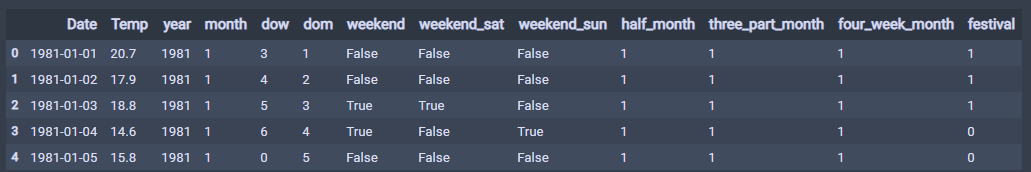有了好的特征才有好的模型的输入，并且对于一年的季度还有一个月的上中下旬，这样用1234表示的定类数据，计算机并不能理解什么意思，123表示上中下，并不意味着下旬就比上旬大2，所以需要转成独热编码的形式显示。
5. 独热编码One-Hot Encoding
独热编码用来将定类数据表示成0-1二进制，便于输入模型中。
比如，计算机并不认识颜色这一列的“红”， “黄”， “绿”三个分类，所以我们用“是不是红色”， “是不是黄色”， “是不是绿色”三列来分开“颜色”这一列特征。
下面开始做独热编码，但是在做独热编码之前，先保存一份数据
before_dummy_df = df2.copy()

# 构造数据集的特征
drop_columns = ['Date', 'Temp']
X_before_dummy = before_dummy_df.drop(drop_columns, axis=1)

# 构造数据集的标签
Y = df['Temp']

"独热向量编码"
columns_to_encoding = ['year', 'month', 'dow', 'dom', 'three_part_month', 'four_week_month']

# 使用pandas的get_dummyise函数对df2指定的列进行独热向量编码
dummy_X = pd.get_dummies(X_before_dummy, columns=columns_to_encoding)

独热编码之后，就会发现数据有了72列特征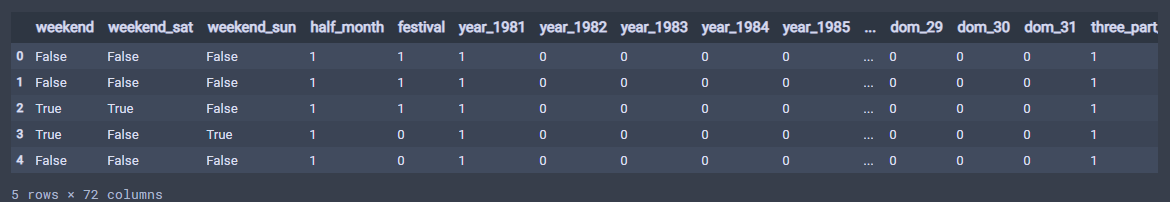我们通过上面，也构造出了X和Y了，到现在我们有两组数据：
没有经过独热向量编码操作的数据X_befor_dummy经过独热向量编码的数据dummy_X
共有标签Y。
有了数据，我们就可以建立模型进行拟合了。
6. 机器学习模型的回归拟合
准备好了数据集，就可以进行回归拟合了，我们先用几个常见的解决回归问题的机器学习模型：
多元线性回归多项式回归岭回归决策树和随机森林支持向量机多层感知机（多层神经网络）
回归模型的评估指标：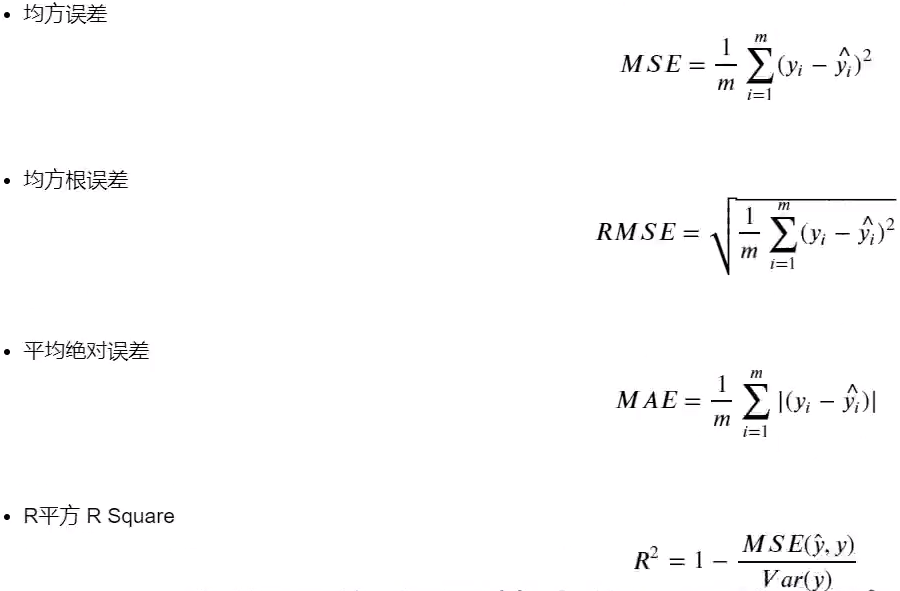6.1 多元线性回归拟合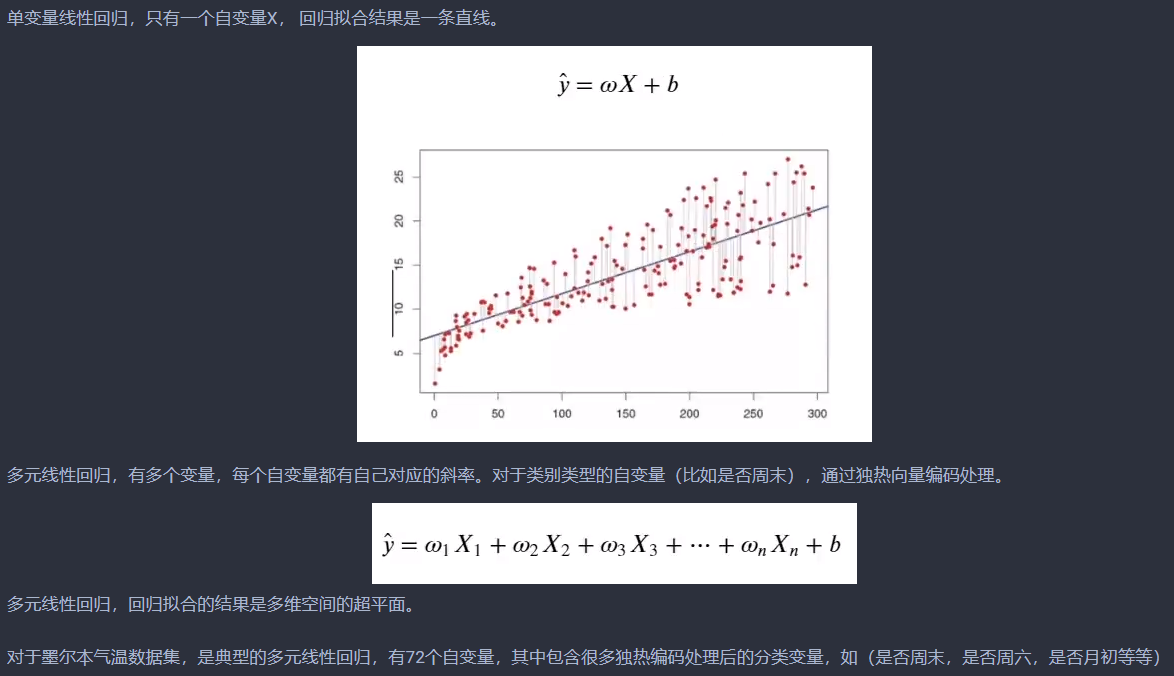代码如下：
from sklearn.model_selection import train_test_split
from sklearn.linear_model import LinearRegression

# 划分训练集和验证集
x_train, x_test, y_train, y_test = train_test_split(dummy_X, Y, test_size=0.2, random_state=1, shuffle=True)

lr_reg = LinearRegression()
lr_reg.fit(x_train, y_train)
print('截距', lr_reg.intercept_)
print('斜率（线性模型中各特征对应的系数）', lr_reg.coef_)

下面把拟合效果进行可视化：
# 绘制气温散点图
df['Temp'].plot(style='k.', figsize=(20, 10))
df.loc[:, '线性回归'] = lr_reg.predict(dummy_X)
plt.plot(df['线性回归'], 'r.')

# 设置坐标文字大小
plt.tick_params(labelsize=20)

# 生成刻度线网格
plt.grid()

结果如下：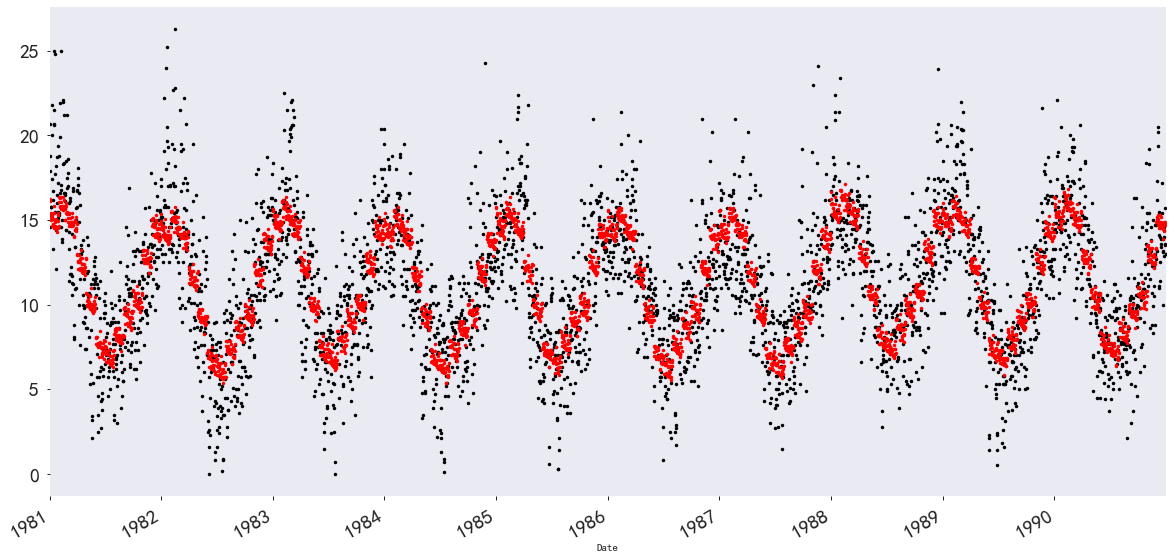6.2 二项多项式回归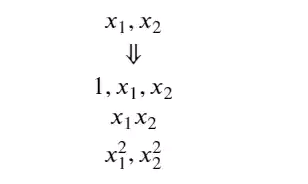代码如下：
from sklearn.preprocessing import PolynomialFeatures

# 构建一个特征处理器poly_reg, 它能将输入特征变成二次的，见上面图
poly_reg = PolynomialFeatures(degree=2)

# 使用构建的二项多项式特征处理器poly_reg处理训练数据dummy_X, 得到包含二次特征的训练集X_poly
X_poly = poly_reg.fit_transform(dummy_X)

解释一下上面的代码：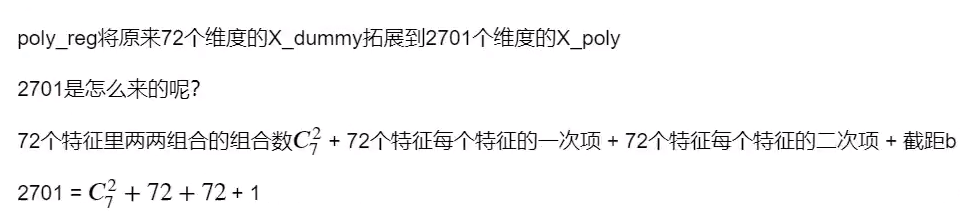构建二次多项式回归模型，将上面构造好的X_poly送进去训练
lin_reg_2 = LinearRegression()
lin_reg_2.fit(X_poly, Y)

# 查看回归方程系数
print('每个维度对应的系数（斜率）：Cofficients: ', lin_reg_2.coef_)

# 查看回归方程截距
print('截距： intercept', lin_reg_2.intercept_)
df.loc[:, '二次多项式回归'] = lin_reg_2.predict(X_poly)

可视化结果：
# 绘制二次多项式回归模型拟合的气温
df.loc[:, '二次多项式回归'] = lin_reg_2.predict(X_poly)
plt.plot(df['二次多项式回归'], 'g*')

# 绘制气温散点图
df['Temp'].plot(style='k.', figsize=(20, 13))

# 设置图例文字大小和图示大小
plt.legend(fontsize=25, markerscale=5)

# 设置坐标文字大小
plt.tick_params(labelsize=25)

# 生成网格
plt.grid()

结果如下：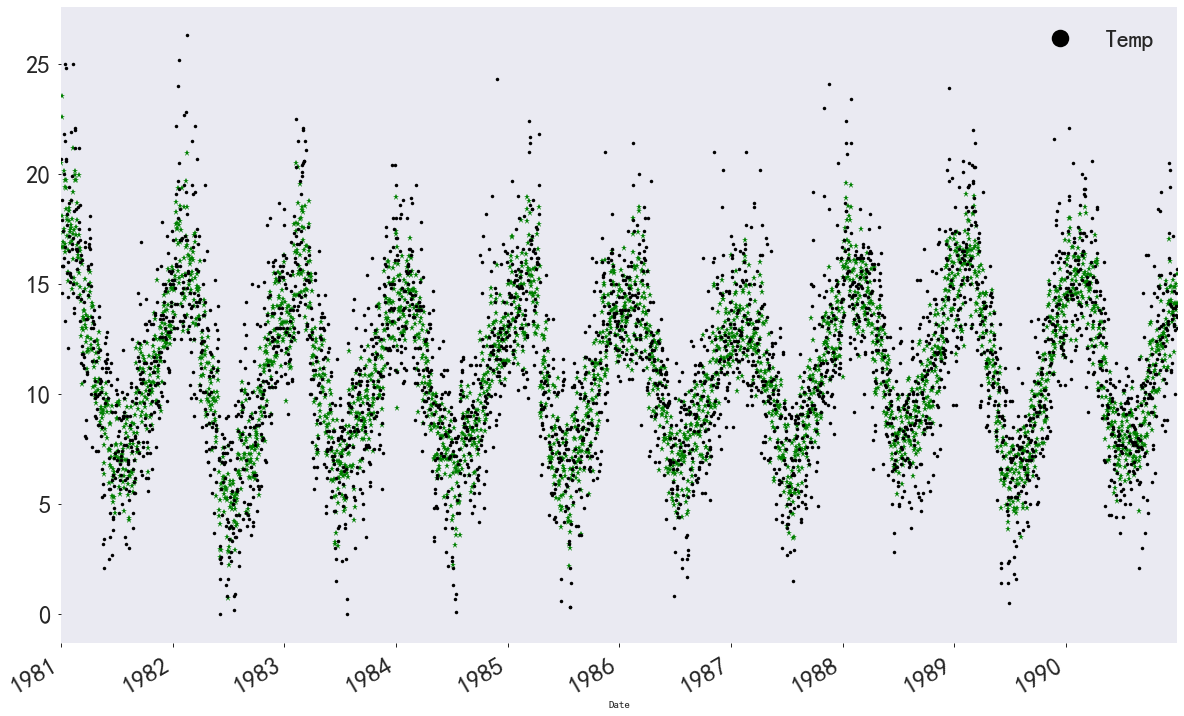三次多项式回归模型：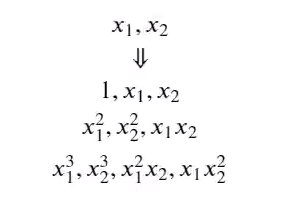# 构建一个特征处理器ploy_reg3, 它能将输入特征变成三次的
ploy_reg3 = PolynomialFeatures(degree=3)

# 使用构建的三次多项式处理训练集dummy_X， 得到包含三次特征的训练集X_poly3
X_poly3 = ploy_reg3.fit_transform(dummy_X)

# 构建三次多项式回归模型并训练
lin_reg_3 = LinearRegression()
lin_reg_3.fit(X_poly3, Y)
df.loc[:, '三次多项式回归'] = lin_reg_3.predict(X_poly3)

这个可视化一下，就会发现这个会过拟合：
# 绘制二次多项式回归模型拟合的气温, 绿色五角星表示
plt.plot(df['二次多项式回归'], 'g*')
# 绘制三次多项式回归模型拟合的气温，红色三角形表示
plt.plot(df['三次多项式回归'], 'r^')

# 绘制气温散点图， 黑色点表示
df['Temp'].plot(style='k.', figsize=(20, 13))

# 设置图例文字大小和图示大小
plt.legend(fontsize=15, markerscale=3)

# 设置坐标文字大小
plt.tick_params(labelsize=25)

# 生成网格
plt.grid()

"""这个三项式的这个明显出现了过拟合现象"""

结果：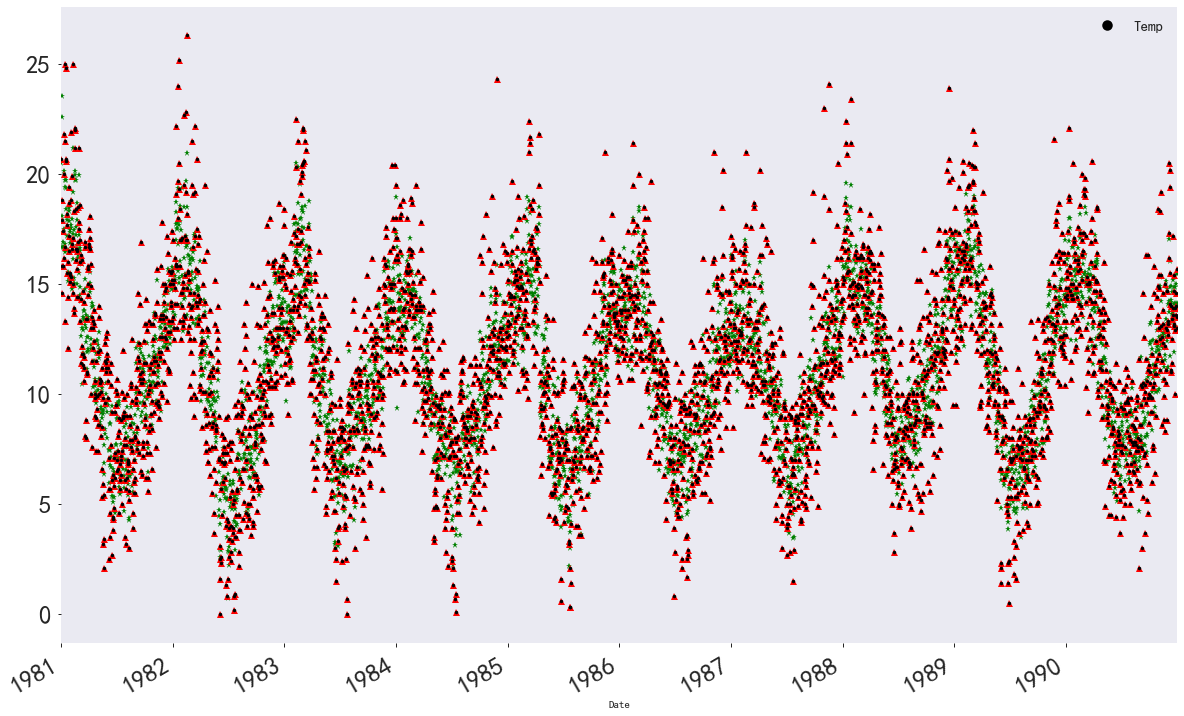三次多项式拟合的点与原始数据完全重合，出现了过拟合。 在机器学习中，我们希望模型能大而化之的学习到数据普遍一般规律，而不是对每一个点死记硬背。 因此，线性回归中，二项多项式回归效果是最好的。
6.3 岭回归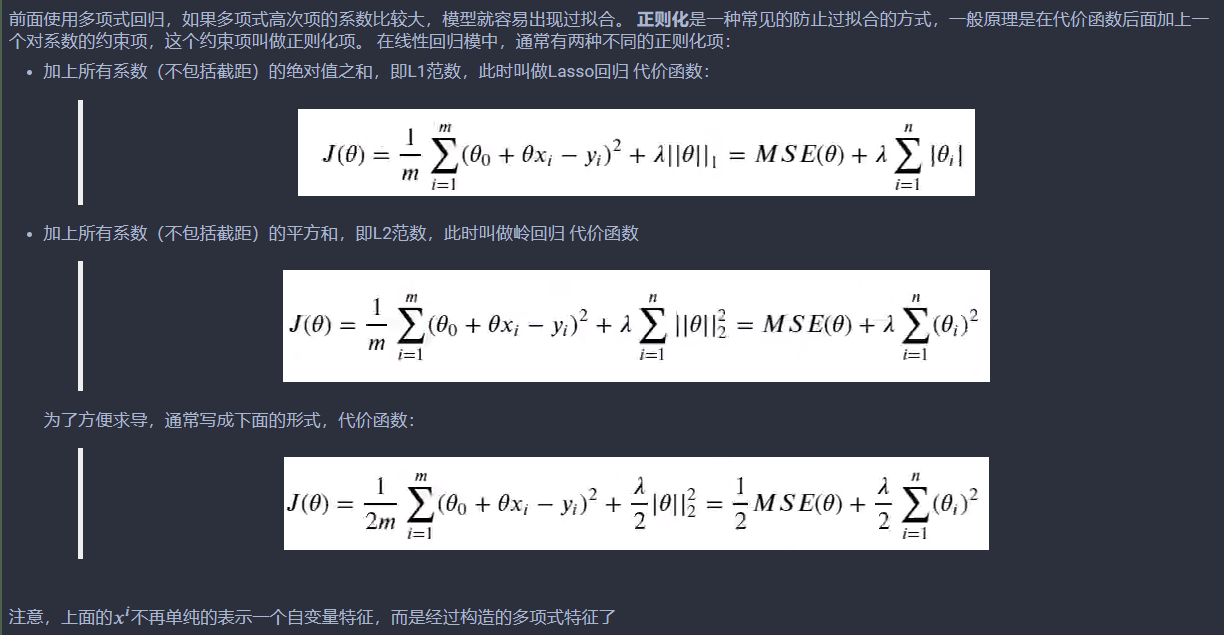代码如下：
from sklearn.model_selection import train_test_split
x_train, x_test, y_train, y_test = train_test_split(dummy_X, Y, test_size=0.2, random_state=1, shuffle=True)

from sklearn.linear_model import RidgeCV

# alphas 表示正则化强度， 类似之前的代价函数中的λ， cv=5表示5折交叉验证
ridge = RidgeCV(alphas=[0.2, 0.5, 0.8], cv=5)
ridge.fit(x_train, y_train)

ridge.score(x_test, y_test)

可视化一下：
# 绘制气温散点图， 用黑色点表示
df['Temp'].plot(style='k.', figsize=(20, 13))

df.loc[:, '岭回归'] = ridge.predict(dummy_X)
plt.plot(df['岭回归'], 'g.')

# 设置图例文字大小和图示大小
plt.legend(fontsize=15, markerscale=3)

# 设置坐标文字大小
plt.tick_params(labelsize=25)

# 生成刻度线网格
plt.grid()

结果：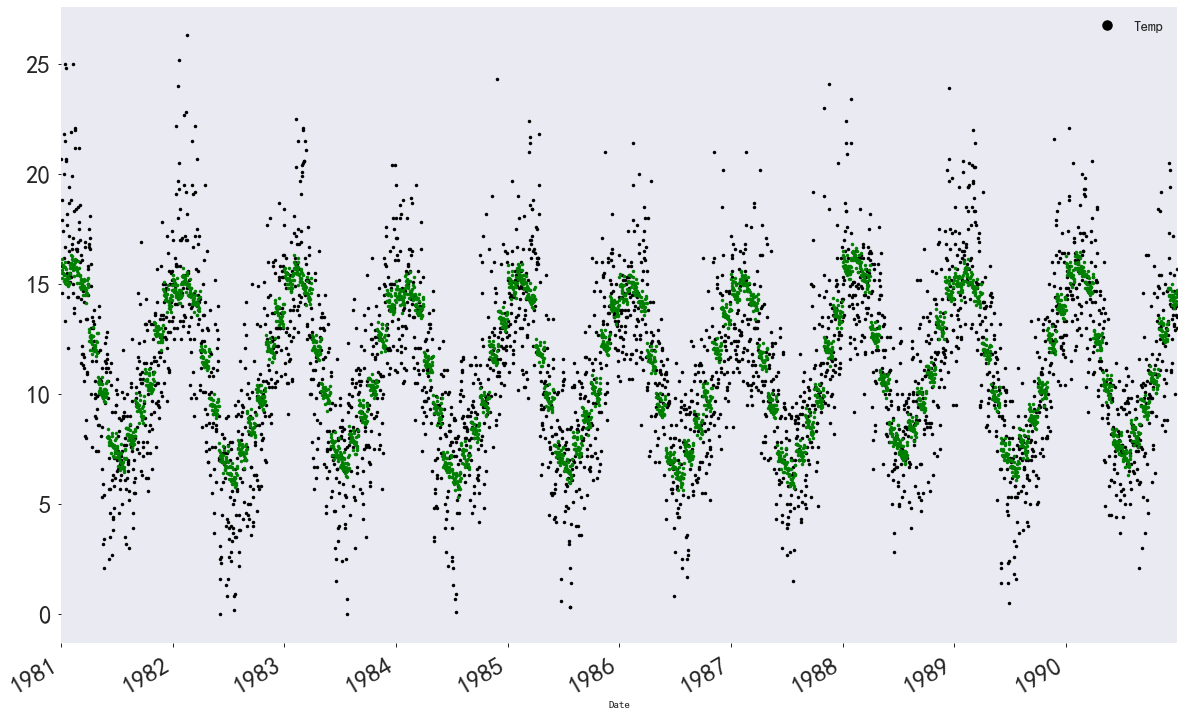6.4 决策树和随机森林
决策树和随机森林的训练数据不需要进行独热向量编码，所以我们可以直接用X_before_dummy。
"""划分训练集和测试集"""
x_train, x_test, y_train, y_test = train_test_split(X_before_dummy, Y, test_size=0.2, random_state=1, shuffle=True)

# 从Python机器学习与数据挖掘工具库sklearn导入随机森林回归器
from sklearn.ensemble import RandomForestRegressor

# 导入网格搜索交叉验证，网格搜索可以让模型参数按我们给定的列表遍历，找到效果最好的模型
# 交叉验证可以充分评估模型的准确性
from sklearn.model_selection import GridSearchCV

# 构造参数字典
param_grid = {
'n_estimators': [5, 10, 20, 50, 100, 200],    # 决策树的个数
'max_depth': [3, 5, 7],   # 最大树身， 树太深会造成过拟合
'max_features': [0.6, 0.7, 0.8, 1]  # 决策树划分时考虑的最大特征数
}

rf = RandomForestRegressor()

# 以随机森林回归器为基础构造网格搜索回归器
grid = GridSearchCV(rf, param_grid=param_grid, cv=3)

# 在训练集上训练
grid.fit(x_train, y_train)

"""选取最优参数对应的模型"""
# 查看效果最好的参数
grid.best_params_
# 指定模型效果最好的参数对应的模型
rf_reg = grid.best_estimator_

# 可视化决策树
from sklearn import tree
import pydotplus
from IPython.display import Image, display

# 从随机森立中选取一棵决策树进行可视化
estimator = rf_reg.estimators_

dot_data = tree.export_graphviz(estimator, out_file=None, filled=True, rounded=True)
graph = pydotplus.graph_from_dot_data(dot_data)
display(Image(graph.create_png()))

6.4.1 特征重要度分析
"""特征重要度分析"""
rf_reg.feature_importances_

print('特征排序')

feature_names = ['year', 'month', 'dow', 'dom', 'weekend', 'weekend_sat', 'weedend_sun', 'half_month',
'three_part_month', 'four_week_month', 'festival']

feature_importances = rf_reg.feature_importances_
indics = np.argsort(feature_importances)[::-1]

for index in indics:
print('features %s (%f)' %(feature_names[index], feature_importances[index]))
plt.figure(figsize=(16, 8))

plt.title('随机森立模型不同特征的重要度')
plt.bar(range(len(feature_importances)), feature_importances[indics], color='b')
plt.xticks(range(len(feature_importances)), np.array(feature_names)[indics], color='b')

可以看到特征重要程度：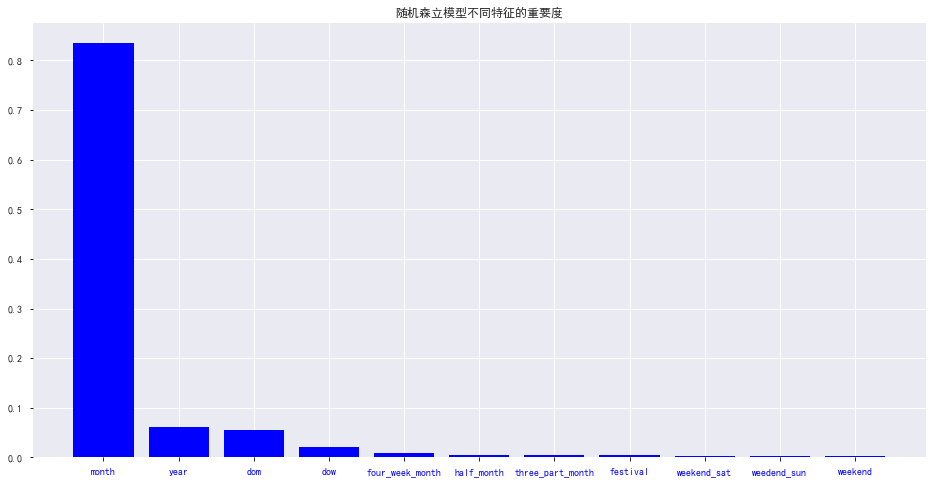6.4.2 可视化回归拟合效果
# 绘制气温散点图
df['Temp'].plot(style='k.', figsize=(20, 15))

# 绘制随机森林模型拟合的气温
df.loc[:, '随机森林'] = rf_reg.predict(X_before_dummy)
plt.plot(df['随机森林'], 'r.')

# 绘制岭回归模型拟合的气温
plt.plot(df['岭回归'], 'g.')

plt.legend(fontsize=15, markerscale=3)

plt.tick_params(labelsize=25)

plt.grid()

结果：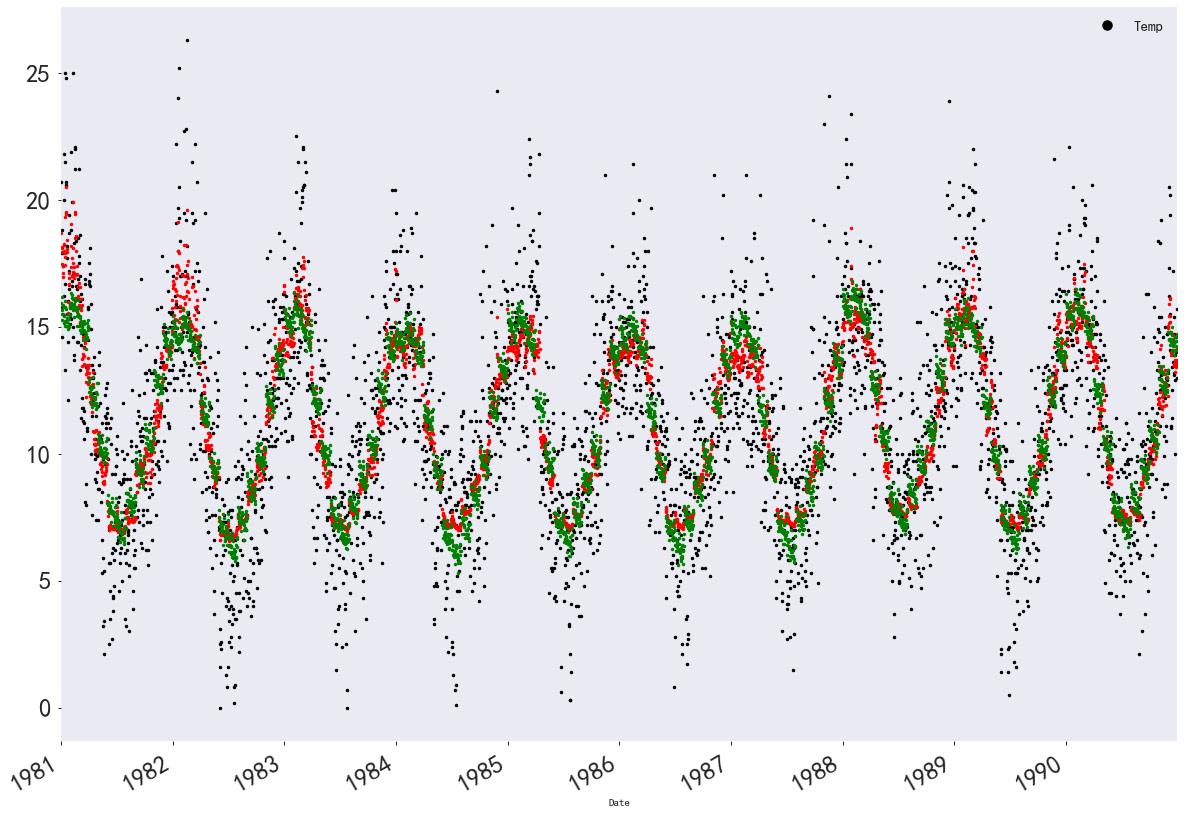6.5 多层神经网络
神经网络对输入特征的幅度很敏感，我们首先需要将输入特征归一化
from sklearn.preprocessing import scale

feature = scale(X_before_dummy)
X_train, X_val, Y_train, Y_val = train_test_split(feature, Y, test_size=0.2, random_state=1, shuffle=True)

"""训练集共有2920条数据， 对应2920天的11个时间特征，标签为对应的气温"""
# 使用Keras模型快速搭建全连接神经网络
from keras.models import Sequential
from keras.layers.core import Dense, Dropout
from keras.optimizers import SGD

model = Sequential()

# 第一层，32个神经元，激活函数是relu， 接收11个特征作为输入

# 第二层，64个神经元，激活函数为relu

# 回归模型的神经网络最后一层不需要有激活函数，直接用一个神经元线性输出结果即可

# 将模型封装好，使用均方误差mse作为损失函数，使用学习率0.001的随机梯度下降算法反向传播，同时平均绝对误差mae监控模型训练效果
model.compile(loss='mse', optimizer=SGD(lr=0.001), metrics=['mae'])

# 训练神经网络, 每一批128个数据， 训练50轮（即过50遍完整的训练集）， 每一轮结束后用验证集评估模型效果
network_history = model.fit(X_train, Y_train, batch_size=128, epochs=50, verbose=1, validation_data=(X_val, Y_val))

绘制结果误差：
"""绘制训练过程的mse误差和mae误差"""
def plot_history(network_history):
plt.figure()
plt.xlabel('Epochs')
plt.ylabel('Loss')
plt.plot(network_history.history['loss'])
plt.plot(network_history.history['val_loss'])
plt.legend(['Training', 'Validation'])

plt.figure()
plt.xlabel('Epochs')
plt.ylabel('Mae')
plt.plot(network_history.history['mean_absolute_error'])
plt.plot(network_history.history['val_mean_absolute_error'])
plt.legend(['Training', 'Validation'])
plt.show()

plot_history(network_history)

模型评估：
model.evaluate(X_val, Y_val, batch_size=10)

结果可视化：
from sklearn.preprocessing import scale
# 绘制气温散点图
df['Temp'].plot(style='k.', figsize=(30, 25))

# 绘制随机森林模型的回归拟合散点图
plt.plot(df['随机森林'], 'r.')

# 绘制岭回归模型的回归拟合散点图
plt.plot(df['岭回归'], 'g.')

# 绘制多层感知神经网络的回归拟合散点图
df.loc[:, "多层神经元"] = model.predict(scale(X_before_dummy))
plt.plot(df['多层神经元'], 'b.')

# 设置图例文字大小和图示大小
plt.legend(fontsize=20, markerscale=5)

# 设置坐标文字大小
plt.tick_params(labelsize=25)

# 生成刻度线网格
plt.grid()

结果如下：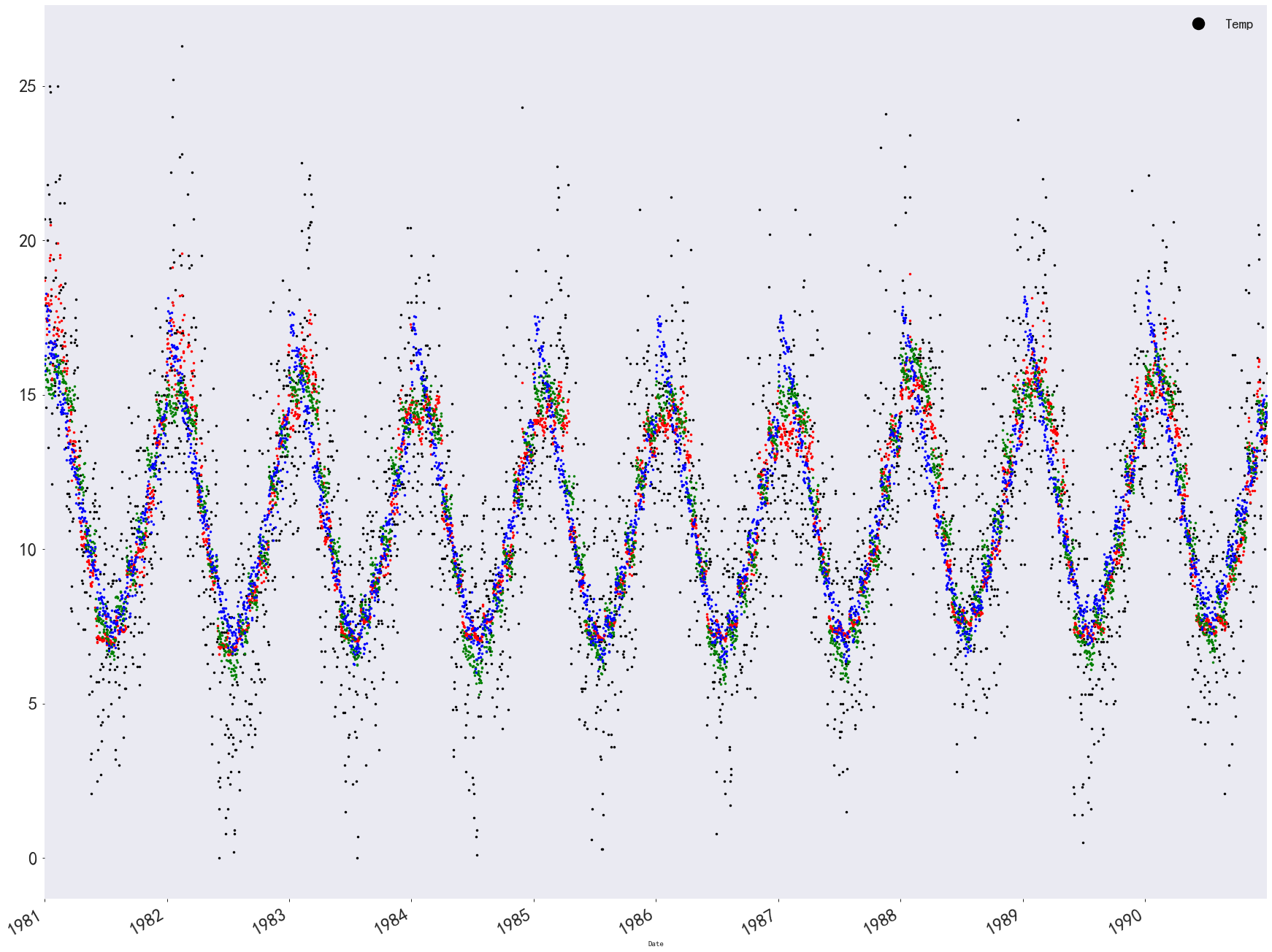7. 将回归拟合的结果文件保存，并比较不同模型的表现
df.to_csv('final_regression.csv', index=True)

result_analyse = result.describe().copy()
# 给result_analyse新构造一行MSE
result_analyse.loc['MSE', :] = 0

# 构造计算均方误差的函数
def MSE(yhat, y):
error = np.array(yhat-y)
error_power = np.power(error, 2)
MSE_error = np.sum(error_power) / len(y)

return MSE_error

# 把result_analyse新构造的MSE行填模型的均方误差
for each in result_analyse.columns:
result_analyse.loc['MSE', each] = MSE(result[each], result['Temp'])
result_analyse

结果如下：最后是一个模型比较的可视化结果：
plt.figure(figsize=(20, 20))
plt.subplot(421)
plt.title('平均值')
result_analyse.loc['mean', :].plot(kind='bar', color='k')

plt.subplot(422)
plt.title('方差')
result_analyse.loc['std', :].plot(kind='bar', color='y')

plt.subplot(423)
plt.title('最小值')
result_analyse.loc['min', :].plot(kind='bar', color='m')

plt.subplot(424)
plt.title('下四分位数')
result_analyse.loc['25%', :].plot(kind='bar', color='c')

plt.subplot(425)
plt.title('中位数')
result_analyse.loc['50%', :].plot(kind='bar', color='r')

plt.subplot(426)
plt.title('上四分位数')
result_analyse.loc['75%', :].plot(kind='bar', color='g')

plt.subplot(427)
plt.title('最大值')
result_analyse.loc['max', :].plot(kind='bar', color='b')

plt.subplot(428)
plt.title('均方误差')
result_analyse.loc['MSE', :].plot(kind='bar', color='deepskyblue')

plt.show()

这里展示一部分结果：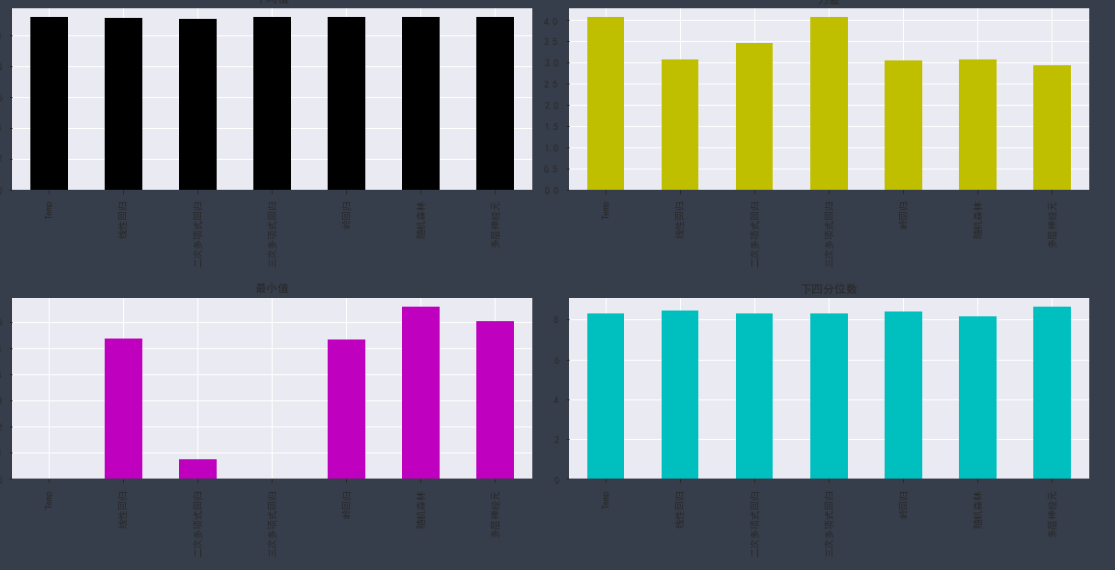8. 总结
这里只是想单纯的整理一个时间序列挖掘模板，方便以后查询使用，毕竟感觉写的还是挺全的，从数据导入，探索，各种格式化，到特征工程，建立各种模型，各种模型的评估等，我觉得在后面的任务中，会有借鉴之处，所以先整理下来。
如果想看详细的讲解视频： 时间序列数据挖掘与机器学习：墨尔本十年气温数据集 如果想获取详细的代码和数据集：https://download.csdn.net/download/wuzhongqiang/12245197
展开全文• 数据挖掘：  数据挖掘从字面上就已经很好理解了，就是从一堆数据中，挖掘出一些有用的信息来的过程。比如说，我们的搜索数据，如果某个地区大部分人都在搜索MH370，表示他们在关注这个事情。我们就可以推荐一些...
数据挖掘：
数据挖掘从字面上就已经很好理解了，就是从一堆数据中，挖掘出一些有用的信息来的过程。比如说，我们的搜索数据，如果某个地区大部分人都在搜索MH370，表示他们在关注这个事情。我们就可以推荐一些安全知识，飞机知识过去。同样的也可能会有蜡烛销售高潮，提前给他们提供便利。这个就是数据挖掘的工作内容。
数据挖掘这些书的主要内容，数据有多少种，什么是有效数据，数据的维度，离散性，相似性。有很大的篇幅是在讲述数据的有效提取等有关数据的性质。剩下的一大部分篇幅就是这些数据的处理。数据的处理，整合，分析，预测等就是机器学习的范围了。
模式识别：
着重物体的识别，基本模式识别都急于图像的，语音的，模式识别里面的数据，大部分都是像素点数据，声音。数据挖掘都是指标类数据，升高，体重，点击率，回复率，关注度等。模式识别的主要内容，比如说，相片里面的这个人是谁，我们手上拿的两种鱼，用电脑识别开，都是模式识别的工作。
图像处理：
图像处理，是模式识别的前期 工作，比如图像上面黯淡无光，就需要进行对比度提升等等。图像处理的书大部分人都看过了，内容就是形态学变换，图像增强，降噪，锐化，直方图分析，图像压缩等等。
做完图像处理后，图像上的内容是什么就交给模式识别了，或者机器学习来进行分类了。
机器视觉：
机器视觉的书籍和图像处理有一部分交叉，可是也有机器视觉也有自己特有的东西，就是坐标定位，三维距离等。这些都是图像处理没有的。从字面上看，机器视觉，主要是针对机器人用摄像头识别类的，它与模式识别比，偏重的是视觉前端，模式识别是后端识别。无论怎么讲，机器人都是需要移动，如何定位当前位置，如何运动都是机器视觉的内容。
人工智能：
人工智能算最宽泛的一种领域了，数据挖掘，模式识别都可以笼统的放到人工智能里面。举例子：文字的理解，表情的分析都是人工智能里面的，人工智能写诗歌，这些都是人工智能。其他的数据挖掘，都是从人工智能里面提取出来的分支而已。人工智能是一个非常大的领域。
至于人工智能的书，都是很宽泛的讲解那些，什么是人工智能，它的历史，有什么难点，文字怎么理解，和人的智能有什么差别等，很理论的东西。
机器学习：
机器学习的书，就是一群数学算法，贝叶斯，均值聚类，神经网络，深度学习，支持向量机等。也是做到分析，推理的核心了。模式识别，最后的识别就是靠它。数据挖掘，最后的分析也是靠它。人工智能，最后的智能现象也要靠它。模式识别，数据挖掘，人工智能都是领域，而最后完成的处理都是机器学习。

基本内容呢？？就是这些了，各位想从事哪个方向呢？？？自己去选择吧。不过我还是分析一下目前的情况吧。
目前的社会情况：
百度，阿里，腾讯和各类金融，互联网公司，大部分的机器学习工程师，都是用于数据挖掘的。也是目前最火爆，待遇最好的方向。所以，偏向于数据挖掘更好一些。
-------------------------------------------------------------------------------------------------------------------

数据挖掘和机器学习的区别和联系，周志华有一篇很好的论述《机器学习与数据挖掘》可以帮助大家理解。数据挖掘受到很多学科领域的影响，其中数据库、机器学习、统计学无疑影响最大。简言之，对数据挖掘而言，数据库提供数据管理技术，机器学习和统计学提供数据分析技术。由于统计学往往醉心于理论的优美而忽视实际的效用，因此，统计学界提供的很多技术通常都要在机器学习界进一步研究，变成有效的机器学习算法之后才能再进入数据挖掘领域。从这个意义上说，统计学主要是通过机器学习来对数据挖掘发挥影响，而机器学习和数据库则是数据挖掘的两大支撑技术。从数据分析的角度来看，绝大多数数据挖掘技术都来自机器学习领域，但机器学习研究往往并不把海量数据作为处理对象，因此，数据挖掘要对算法进行改造，使得算法性能和空间占用达到实用的地步。同时，数据挖掘还有自身独特的内容，即关联分析。
而模式识别和机器学习的关系是什么呢，传统的模式识别的方法一般分为两种：统计方法和句法方法。句法分析一般是不可学习的，而统计分析则是发展了不少机器学习的方法。也就是说，机器学习同样是给模式识别提供了数据分析技术。
至于，数据挖掘和模式识别，那么从其概念上来区分吧，数据挖掘重在发现知识，模式识别重在认识事物。
机器学习的目的是建模隐藏的数据结构，然后做识别、预测、分类等。
因此，机器学习是方法，模式识别是目的
展开全文• 从使用的广义角度上看，数据挖掘常用分析方法主要有分类、聚类、估值、预测、关联规则、可视化等。从数据挖掘算法所依托的数理基础角度归类，目前数据挖掘算法主要分为三大类:机器学习方法、统计方法与神经网络方法...
 一. 分类  二. 聚类  三. 关联规则  四 时间序列预测

1. 分类
在数据挖掘的发展过程中，由于数据挖掘不断地将诸多学科领域知识与技术融入当中，因此，目前数据挖掘方法与算法已呈现出极为丰富的多种形式。从使用的广义角度上看，数据挖掘常用分析方法主要有分类、聚类、估值、预测、关联规则、可视化等。从数据挖掘算法所依托的数理基础角度归类，目前数据挖掘算法主要分为三大类:机器学习方法、统计方法与神经网络方法。机器学习方法分为决策树、基于范例学习、规则归纳与遗传算法等:统计方法细分为回归分析、时间序列分析、关联分析、聚类分析、模糊集、粗糙集、探索性分析、支持向量机与最近邻分析等:神经网络方法分为前向神经网络、自组织神经网络、感知机、多层神经网络、深度学习等。在具体的项目应用场景中通过使用上述这些特定算法，可以从大数据中整理并挖掘出有价值的所需数据，经过针对性的数学或统计模型的进一步解释与分析， 提取出隐含在这些大数据中的潜在的规律、规则、知识与模式口。下面介绍数据挖据中经常使用的分类、聚类、关联规则与时间序列预测等相关概念。  数据挖掘方法中的一种重要方法就是分类，在给定数据基础上构建分类函数或分类模型，该函数或模型能够把数据归类为给定类别中的某一种类别，这就是分类的概念。在分类过程中，通常通过构建分类器来实现具体分类，分类器是对样本进行分类的方法统称。一般情况下，分类器构建需要经过以下4步:①选定包含正、负样本在内的初始样本集，所有初始样本分为训练与测试样本;②通过针对训练样本生成分类模型:③针对测试样本执行分类模型，并产生具体的分类结果:④依据分类结果，评估分类模型的性能。在评估分类模型的分类性能方面，有以下两种方法可用于对分类器的错误率进行评估:①保留评估方法。通常采用所有样本集中的2/3部分样本作为训练集，其余部分样本作为测试样本，也即使用所有样本集中的2/3样本的数据来构造分类器，并采用该分类器对测试样本分类，评估错误率就是该分类器的分类错误率。这种评估方法具备处理速度快的特点，然而仅用2/3 样本构造分类器，并未充分利用所有样本进行训练。②交叉纠错评估方法。该方法将所有样本集分为N个没有交叉数据的子集，并训练与测试共计N次。在每一次 训练与测试过程中，训练集为去除某- 个子集的剩余样本，并在去除的该子集上进行N次测试，评估错误率为所有分类错误率的平均值。一般情况下，保留评估方法用于最初试验性场景，交叉纠错法用于建立最终分类器。

2. 聚类
随着科技的进步，数据收集变得相对容易，从而导致数据库规模越来越庞大，例如，各类网上交易数据、图像与视频数据等，数据的维度通常可以达到成百上千维。在自然社会中，存在大量的数据聚类问题，聚类也就是将抽象对象的集合分为相似对象组成的多个类的过程，聚类过程生成的簇称为一组数据对象的集合。聚类源于分类，聚类又称为群分析，是研究分类问题的另种统计计算方法， 但聚类又不完全等同于分类同。聚类与分类的不同点在于:聚类要求归类的类通常是未知的，而分类则要求事先已知多个类。对于聚类问题，传统聚类方法已经较为成功地解决了低维数据的聚类，但由于大数据处理中的数据高维、多样与复杂性，现有的聚类算法对于大数据或高维数据的情况下，经常面临失效的窘境。受维度的影响，在低维数据空间表现良好的聚类方法，运用在高维空间上却无法获得理想的聚类效果。在针对高维数据进行聚类时，传统聚类方法主要面临两个问题:①相对低维空间中的数据，高维空间中数据分布稀疏，传统聚类方法通常基于数据间的距离进行聚类，因此，在高维空间中采用传统聚类方法难以基于数据间距离来有效构建簇。②高维数据中存在大量不相关的属性，使得在所有维中存在簇的可能性几乎为零。目前，高维聚类分析已成为聚类分析的个重要研究方向，也是聚类技术的难点与挑战性的工作。

3. 关联规则
关联规则属于数据挖掘算法中的一类重要方法，关联规则就是支持度与置信度分别满足用户给定阈值的规则4。所谓关联，反映一个事件与其他事件间关联的知识。支持度揭示了A和B同时出现的频率。置信度揭示了B出现时，A有多大的可能出现。关联规则最初是针对购物篮分析问题提出的，销售分店经理想更多了解顾客的购物习惯，尤其想获知顾客在一次购物时会购买哪些商品。通过发现顾客放入购物篮中不同商品间的关联，从而分析顾客的购物习惯。关联规则的发现可以帮助销售商掌握顾客同时会频繁购买哪些商品，从而有效帮助销售商开发良好的营销手段。1993 年，R.Agrawal首次提出挖掘顾客交易数据中的关联规则问题，核心思想是基于二阶段频繁集的递推算法。起初关联规则属于单维、单层及布尔关联规则，例如，典型的Aprior 算法。在工作机制上，关联规则包含两个主要阶段:第1阶段先从资料集合中找出所有的高频项目组，第2阶段由高频项目组中产生关联规则。随着关联规则的不断发展，目前关联规则中可以处理的数据分为单维和多维数据。针对单维数据的关联规则中，只涉及数据的一一个维，如客户购买的商品:在针对多维数据的关联规则中，处理的数据涉及多个维。总体而言，单维关联规则处理单个属性中的一些关系，而多维关联规则处理各属性间的关系。

4. 时间序列预测
通常将统计指标的数值按时间顺序排列所形成的数列，称为时间序列。时间序列预测法是一种历史引申预测法，也即将时间数列所反映的事件发展过程进行引申外推，预测发展趋势的一种方法。时间序列分析是动态数据处理的统计方法，主要基于数理统计与随机过程方法，用于研究随机数列所服从的统计学规律，常用于企业经营、气象预报、市场预测、污染源监控、地震预测、农林病虫灾害预报、天文学等方面。时间序列预测及其分析是将系统观测所得的时数据，通过 参数估计与曲线拟合来建立合理数学模型的方法，包含谱分析与自相关分析在内的一系列统计分析理论， 涉及时间序列模型的建立、推断、最优预测、非线性控制等原理。时间序列预测法可用于短期、中期和长期预测，依据所采用的分析方法，时间序列预测又可以分为简单序时平均数法、移动平均法、季节性预测法、趋势预测法、指数平滑法等方法。
展开全文算法 分类 聚类
•   本文运用数据挖掘技术对市财政收入进行分析，挖掘其中的隐藏的运行模式，并对未来两年的财政收入进行预测，希望能够帮助政府合理地控制财政收支，优化财政建设，为制定相关决策提供依据。定义数据挖掘目标如下：...
• 第一次正经的去参加数据挖掘的比赛，从第一赛季开始到第二赛季结束，完整地经历了整个流程，每天提出新想法，学习新的方法，然后用编程的方法去实现，看着自己的MAPE一天天的下降，那种感觉也是很棒的。觉得付出了很...
• 挖掘目的用数字图像处理部分来作为数据挖掘分析的数据源，按水色判断水质分类的数据以及用数码相机按照标准进行水色采集的图像数据，利用图像处理技术，通过水色图像实现水质的自动评价。分析方法水样图像维度过大，...颜色矩 支持向量机
• 数据挖掘：  数据挖掘从字面上就已经很好理解了，就是从一堆数据中，挖掘出一些有用的信息来的过程。比如说，我们的搜索数据，如果某个地区大部分人都在搜索MH370，表示他们在关注这个事情。我们就可以推荐一些安全...机器学习 模式识别 神经网络
• ## 数据挖掘笔记

千次阅读 2015-07-22 13:18:04数据集成 可视化 操作
• ## 数据挖掘

千次阅读 2011-12-08 15:54:01
什么是数据挖掘数据挖掘的起源数据挖掘能做什么数据挖掘中的关联规则数据挖掘和在线分析处理（OLAP）数据挖掘，机器学习和统计软硬件发展对数据挖掘的影响数据挖掘相关的10个问题 展开 　   数据挖掘 ...数据仓库 数据库 算法
• 用在挖掘预测领域： 应用场景：店铺销量预测、量化投资、广告推荐、企业客户分类、SQL语句安全检测分类… 用在图像领域： 应用场景：街道交通标志检测、人脸识别等等 用在自然语言处理领域： 应用场景：文本...
• 预测类模型根据被解释变量的度量类型，分为对连续变量建模的回归，对分类变量建模的分类器，其中以二分类器为主。这里的回归不是仅有线性回归，还有回归决策树、回归神经网络，甚至最近邻域（KNN）和支持向量机（SVM...算法
• ## 数据挖掘简介

千次阅读 2017-11-30 10:02:51
1.什么是数据挖掘？ 从大量数据(含文本)中挖掘出隐含的、未知的、对决策有潜在价值的关系、模式和趋势，并用这些知识和规则建立用于决策支持的模型，提供预测性决策支持的关系、工具和过程； 2.数据挖掘能做什么...
• 基于分析型CRM数据挖掘的移动客户流失预测模型分析与设计，李易桥，，本文通过研究人脑对视觉信息的处理过程，提出了一种新的自然图像压缩方法，该方法。本文在对目前我国电信企业新的竞争格局分析的客户流失
• 一、数据挖掘、知识发现的概念 数据挖掘 Data Mining ：是指从海量的数据中通过相关的算法发现隐藏在数据中的规律和知识的过程。 知识发现：数据挖掘是知识发现中的一个步骤。当提到“数据挖掘”时，通常情况下要...
• 通过在数据挖掘系统上执行算法做的预测和分类的准确率，我们可以判断系统中的算法是否合理，数据采集是否全面以及数据预处理工作是否完善。 性能 该系统能否在我们需要的商业平台运行；软件的架构是否能连接...大数据
• ## 数据挖掘报告

万次阅读 热门讨论 2010-04-10 10:03:00
研究方向前沿读书报告数据挖掘技术的算法与应用 目录第一章 数据仓库... 51.1 概论... 51.2 数据仓库体系结构... 61.3 数据仓库规划、设计与开发... 71.3.1 确定范围... 71.3.2 环境评估... 71.3.3 分析... 71.3.4 ...算法 数据仓库 数据库
• 第2章软件介绍 第3章数据探索 第4章数据预处理 第5章 挖掘建模之离群点检测聚类分析关联规则分类与预测 第6章电力窃漏电用户自动识别 第7章航空公司客户价值分析 ...第16章基于MATLAB的数据挖掘二次开发
• ## 数据挖掘十大算法之CART详解

万次阅读 多人点赞 2016-11-23 11:47:57
在2006年12月召开的 IEEE 数据挖掘国际会议上，与会的各位专家选出了当时的十大数据挖掘算法（ top 10 data mining algorithms ），本博客的十大数据挖掘算法系列文章已经介绍了其中的六个，本文主要讨论CART，即...机器学习 决策树
• 这个岗位叫法很多，算法岗，数据挖掘岗，机器学习岗，基础研究等等，总结一下从16年9月校招的面试情况。百度：师姐给我内推的，一面聊了2个半小时，基本在写代码。用MapReduce写好友推荐，在一堆单词里面找出现次数...算法岗面试 机器学习面试 算法面试
• 该批数据是存储再postgresql里面，是从某售房网站上爬下来的，以数据库中的一列字段作为存储数据，该列字段是以json的数据形式存储的，这里跟我打开了一个新大门，数据库能存储json数据格式的数据，而且postgresql......